Jekyll2023-05-10T08:55:28+00:00https://decentdescent.org/feed.xmlDecent DescentA slow descent into the AIbyss, by [Greg Yang](https://twitter.com/thegregyang) and friends.Tuning GPT-3 on a Single GPU2022-03-08T14:00:00+00:002022-03-08T14:00:00+00:00https://decentdescent.org/tp5Cross-posted from Microsoft Research BlogGreat scientific achievements cannot be made by trial and error alone. Every launch in the space program is underpinned by centuries of fundamental research in aerodynamics, propulsion, and celestial bodies. In the same way, when it comes to building large-scale AI systems, fundamental research forms the theoretical insights that drastically reduce the amount of trial and error necessary and can prove very cost-effective.

In this post, we relay how our fundamental research enabled us, for the first time, to tune enormous neural networks that are too expensive to train more than once. We achieved this by showing that a particular parameterization preserves optimal hyperparameters across different model sizes. This is the µ-Parametrization (or µP, pronounced “myu-P”) that we introduced in a previous paper, where we showed that it uniquely enables maximal feature learning in the infinite-width limit. In collaboration with researchers at OpenAI, we verified its practical advantage on a range of realistic scenarios, which we describe in our new paper, “Tensor Programs V: Tuning Large Neural Networks via Zero-Shot Hyperparameter Transfer.”

By greatly reducing the need to guess which training hyperparameters to use, this technique can accelerate research on enormous neural networks, such as GPT-3 and potentially larger successors in the future. We also released a PyTorch package that facilitates the integration of our technique in existing models, available on the project GitHub page or by simply running pip install mup.

“µP provides an impressive step toward removing some of the black magic from scaling up neural networks. It also provides a theoretically backed explanation of some tricks used by past work, like the T5 model. I believe both practitioners and researchers alike will find this work valuable.”

— Colin Raffel, Assistant Professor of Computer Science, University of North Carolina at Chapel Hill and co-creator of T5

# Scaling the Initialization Is Easy, But Scaling Training Is Hard

Large neural networks are hard to train partly because we don’t understand how their behavior changes as their size increases. Early work on deep learning, such as by Glorot & Bengio and He et al., generated useful heuristics that deep learning practitioners widely use today. In general, these heuristics try to keep the activation scales consistent at initialization. However, as training starts, this consistency breaks at different model widths, as illustrated on the left in Figure 1.

Unlike at random initialization, behavior during training is much harder to mathematically analyze. Our goal is to obtain a similar consistency so that as model width increases, the change in activation scales during training stay consistent and similar to initialization to avoid numerical overflow and underflow. Our solution, µP, achieves this goal, as seen on the right in Figure 1, which shows the stability of network activation scales for the first few steps of training across increasing model width.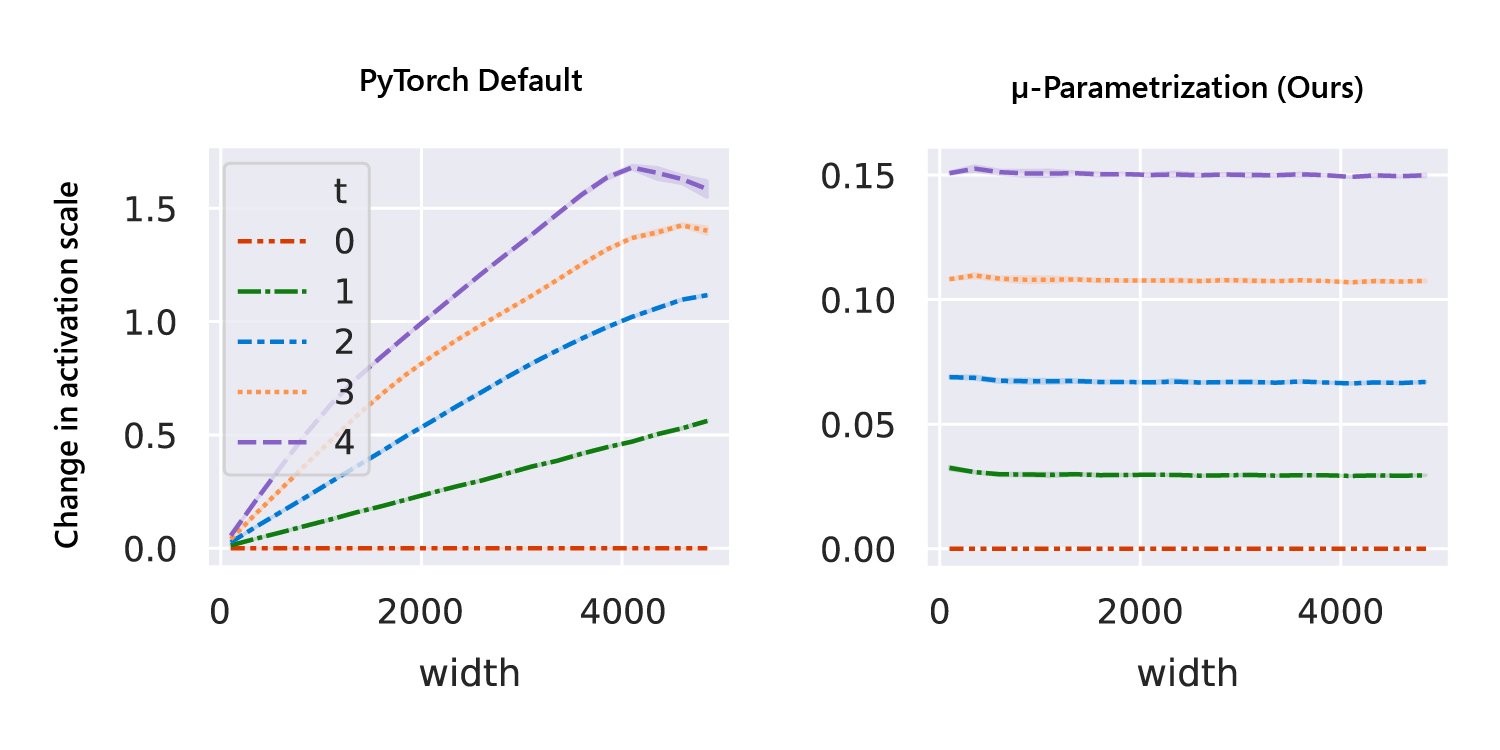Figure 1: In the default parameterization in PyTorch, the graph on the left, the activation scales diverge in width after one step of training. But in µP, the graph on the right, the activation scales change by a consistent amount regardless of width for any training step. The y-axis shows the change of network activation scales on a fixed input after t=0, 1, 2, 3, and 4 steps of training as the width of the model varies, which is shown along the x-axis.

Our parameterization, which maintains this consistency during training, follows two pieces of crucial insight. First, gradient updates behave differently from random weights when the width is large. This is because gradient updates are derived from data and contain correlations, whereas random initializations do not. Therefore, they need to be scaled differently. Second, parameters of different shapes also behave differently when the width is large. While we typically divide parameters into weights and biases, with the former being matrices and the latter vectors, some weights behave like vectors in the large-width setting. For example, the embedding matrix in a language model is of size vocabsize x width. While the width tends to infinity, vocabsize stays constant and finite. During matrix multiplication, the difference in behavior between summing along a finite dimension and an infinite one cannot be more different.

These insights, which we discuss in detail in a previous blog post, motivated us to develop µP. In fact, beyond just keeping the activation scale consistent throughout training, µP ensures that neural networks of different and sufficiently large widths behave similarly during training such that they converge to a desirable limit, which we call the feature learning limit

# A Theory-Guided Approach to Scaling Width

Our theory of scaling enables a procedure to transfer training hyperparameters across model sizes. If, as discussed above, µP networks of different widths share similar training dynamics, they likely also share similar optimal hyperparameters. Consequently, we can simply apply the optimal hyperparameters of a small model directly onto a scaled-up version. We call this practical procedure µTransfer. If our hypothesis is correct, the training loss-hyperparameter curves for µP models of different widths would share a similar minimum.

Conversely, our reasoning suggests that no scaling rule of initialization and learning rate other than µP can achieve the same result. This is supported by the animation below. Here, we vary the parameterization by interpolating the initialization scaling and the learning rate scaling between PyTorch default and µP. As shown, µP is the only parameterization that preserves the optimal learning rate across width, achieves the best performance for the model with width 213 = 8192, and where wider models always do better for a given learning rate—that is, graphically, the curves don’t intersect.Figure 2: On the left, we train multilayer perceptrons (MLPs) of different widths (which correspond to the curves of different colors and patterns) with different learning rates (shown along the x-axis) on CIFAR10 and plot the training loss along the y-axis. On the right, the 2D plane of parameterizations is formed by interpolation of 1) the initialization scaling between PyTorch default and µP (x-axis), and 2) the learning rate scaling between PyTorch default and µP (y-axis). On this plane, PyTorch default is represented by (0, 0) and µP by (1, 1). The width-256 (log2(width) = 8) model is the same across all frames (except for random seed), but we widen models according to the parameterization represented by the dot on the right.

Building on the theoretical foundation of Tensor Programs, µTransfer works automatically for advanced architectures, such as Transformer and ResNet. It can also simultaneously transfer a wide range of hyperparameters. Using Transformer as an example, we demonstrate in Figure 3 how the optima of key hyperparameters are stable across widths.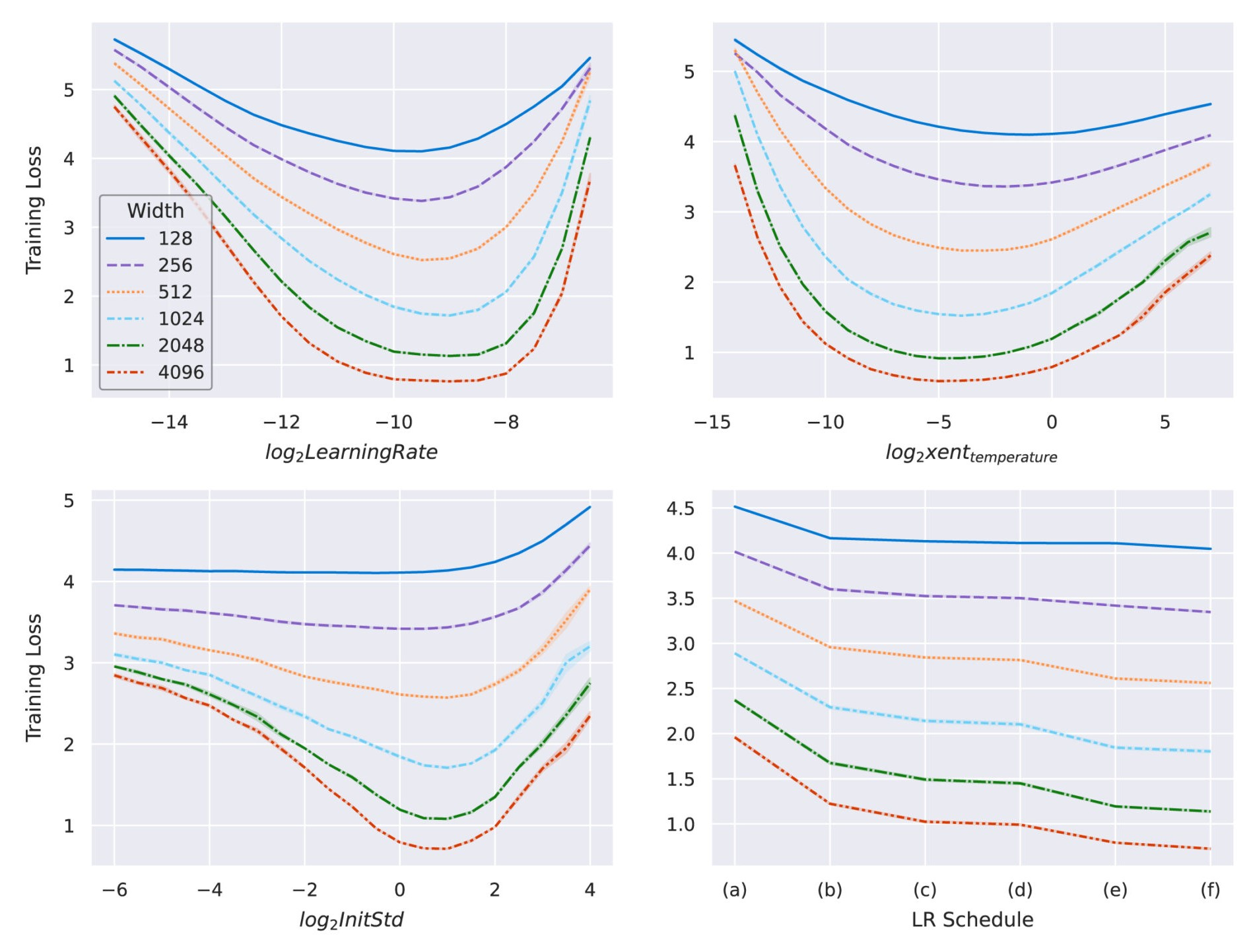Figure 3: Transformers of different widths parameterized in µP and trained on WikiText-2. As we increase model width, the optimal learning rate, cross-entropy temperature, initialization scale, and learning rate schedule remain stable. We can meaningfully predict the optimal hyperparameters of a wider network by looking at those of a narrow one. In plot on the lower right, we tried the following learning rate schedules: (a) linear decay, (b) StepLR @ [5k, 8k] with a decay factor of 0.1, (c) StepLR @ [4k, 7k] with a decay factor of 0.3, (d) cosine annealing,(e) constant, and (f) inverse square-root decay.

“I am excited about µP advancing our understanding of large models. µP’s principled way of parameterizing the model and selecting the learning rate make it easier for anybody to scale the training of deep neural networks. Such an elegant combination of beautiful theory and practical impact.”

— Johannes Gehrke, Technical Fellow, Lab Director of Research at Redmond, and CTO and Head of Machine Learning for the Intelligent Communications and Conversations Cloud (IC3)

# Beyond Width: Empirical Scaling of Model Depth and More

Modern neural network scaling involves many more dimensions than just width. In our work, we also explore how µP can be applied to realistic training scenarios by combining it with simple heuristics for nonwidth dimensions. In Figure 4, we use the same transformer setup to show how the optimal learning rate remains stable within reasonable ranges of nonwidth dimensions. For hyperparameters other than learning rate, see Figure 19 in our paper.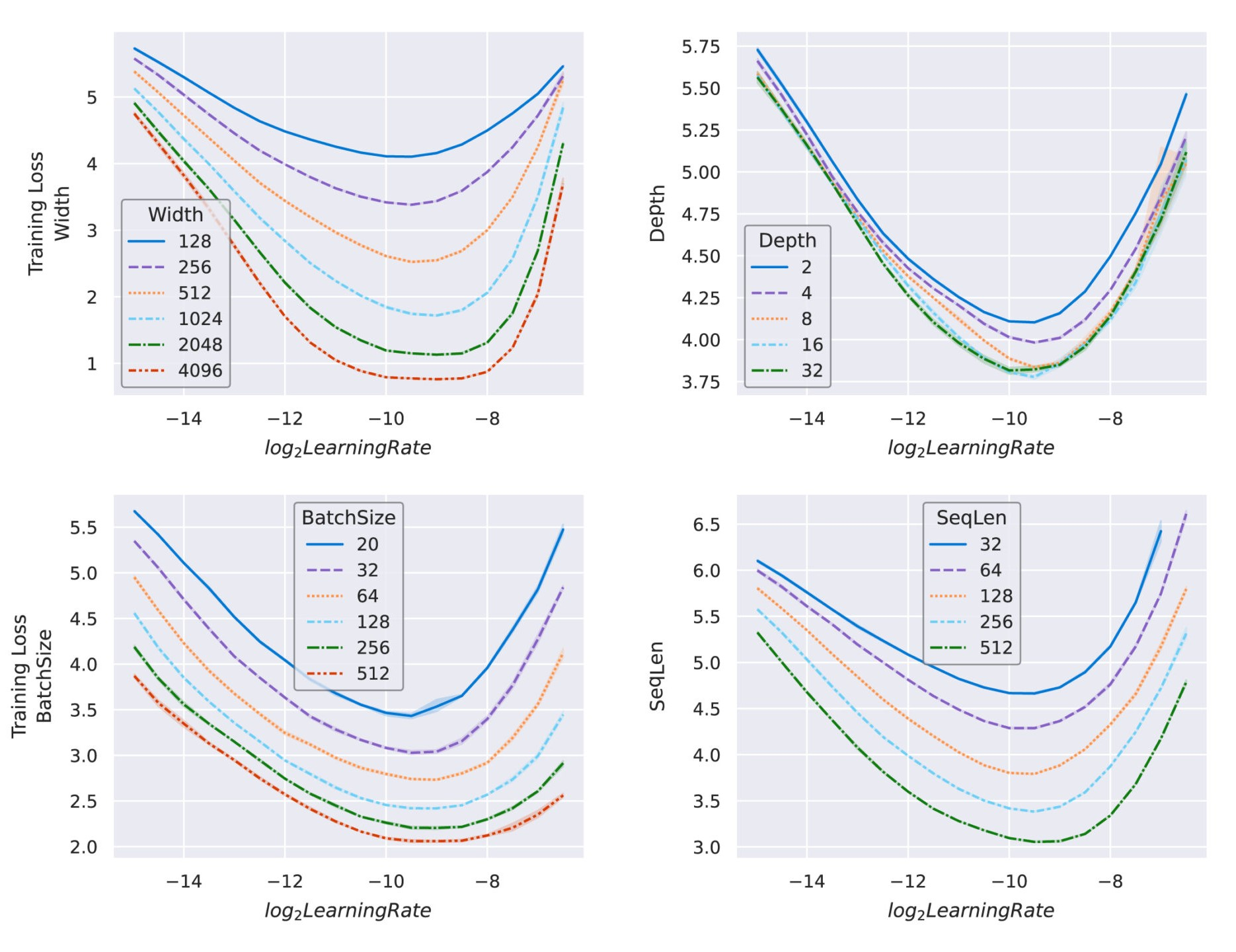Figure 4: Transformers of different sizes parameterized in µP and trained on Wikitext-2. Not only does the optimal learning rate transfer across width, as shown in Figure 3, it also empirically transfers across other scale dimensions—such as depth, batch size, and sequence length—across the ranges we tested here. This means we can combine our theoretically motivated transfer across width with the empirically verified one across other scale dimensions to obtain the practical procedure, µTransfer, to tune hyperparameters indirectly on a small model and transfer to a large one.

# Testing µTransfer

Now that we have verified the transfer of individual hyperparameters, it is time to combine them in a more realistic scenario. In Figure 5, we compare µTransfer, which transfers tuned hyperparameters from a small proxy model, with directly tuning the large target model. In both cases, the tuning is done via random search. Figure 5 illustrates a Pareto frontier of the relative tuning compute budget compared with the tuned model quality (BLEU score) on IWSLT14 De-En, a machine translation dataset. Across all compute budget levels, µTransfer is about an order of magnitude (in base 10) more compute-efficient for tuning. We expect this efficiency gap to dramatically grow as we move to larger target model sizes.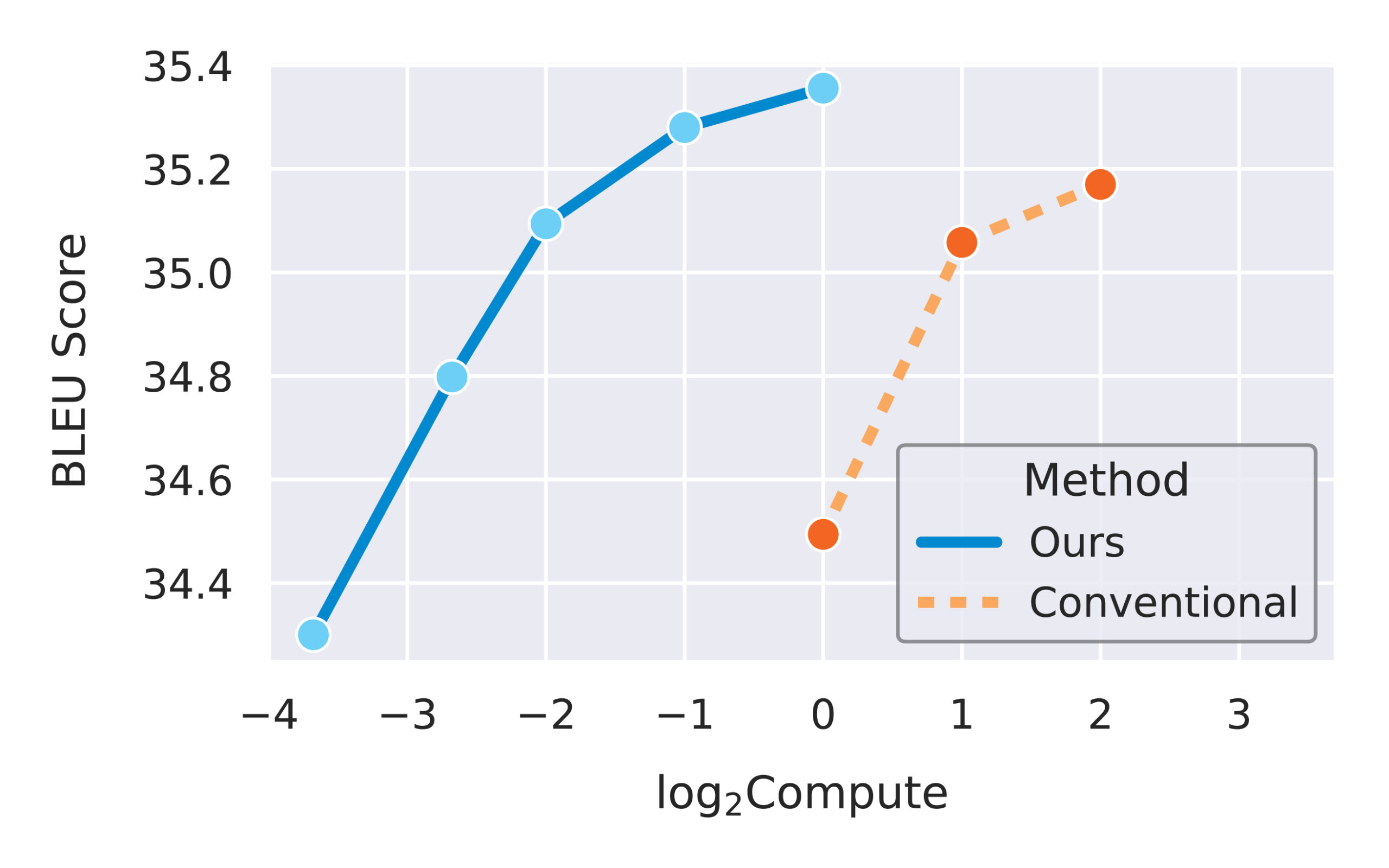Figure 5: Across different tuning budgets, µTransfer dominates the baseline method of directly tuning the target model. As we train larger target models with billions of parameters, we expect the performance gap to widen, since the proxy model can remain small while still meaningfully predicting the optimal hyperparameters, as shown in Figures 3 and 4.

# A Glimpse of the Future: µP + GPT-3

Before this work, the larger a model was, the less well-tuned we expected it to be due to the high cost of tuning. Therefore, we expected that the largest models could benefit the most from µTransfer, which is why we partnered with OpenAI to evaluate it on GPT-3.

After parameterizing a version of GPT-3 with relative attention in µP, we tuned a small proxy model with 40 million parameters before copying the best hyperparameter combination to the 6.7-billion parameter variant of GPT-3, as prescribed by µTransfer. The total compute used during this tuning stage was only 7 percent of the compute used in the pretraining of the final 6.7-billion model. This µTransferred model outperformed the model of the same size (with absolute attention) in the original GPT-3 paper. In fact, it performs similarly to the model (with absolute attention) with double the parameter count from the same paper, as shown in Figure 6.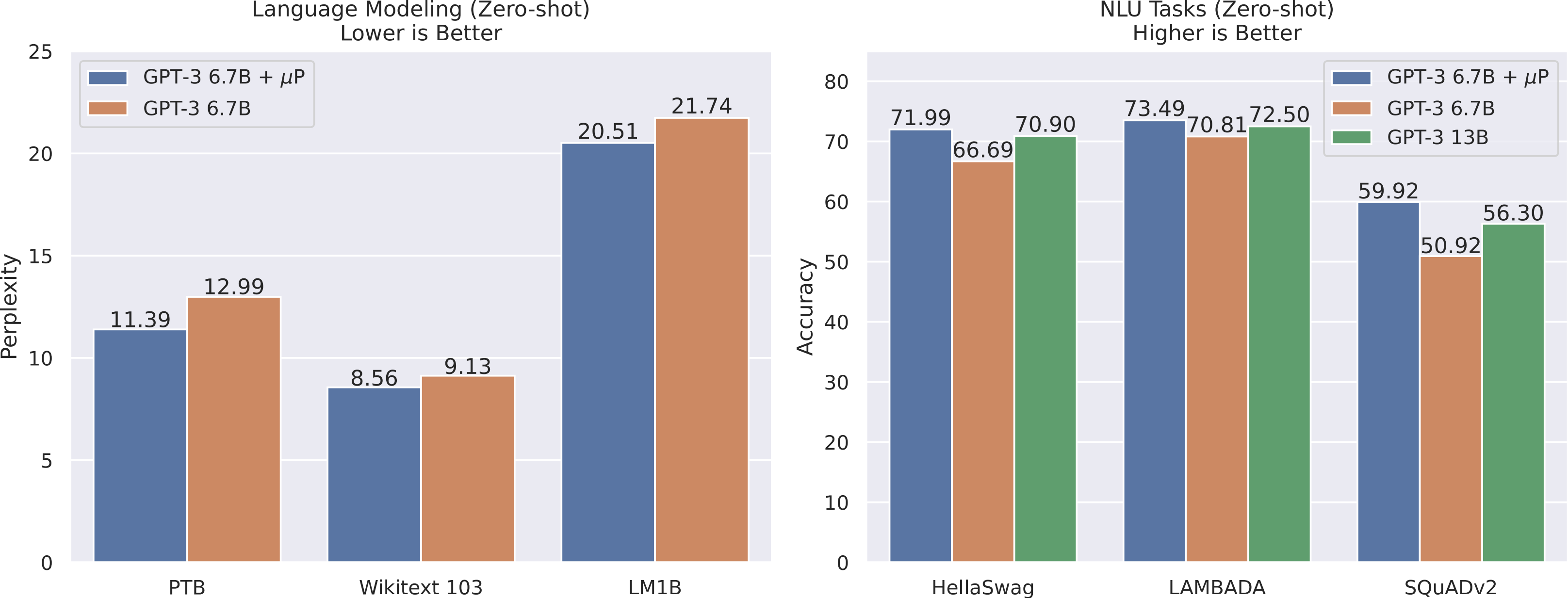Figure 6: We applied µTransfer to GPT-3 6.7-billion parameter model with relative attention and obtained better results than the baseline with absolute attention used in the original GPT-3 paper, all while only spending 7 percent of the pretraining compute budget on tuning. The performance of this µTransfer 6.7-billion model is comparable to that of the 13-billion model (with absolute attention) in the original GPT-3 paper.

# Implications for Deep Learning Theory

As shown previously, µP gives a scaling rule which uniquely preserves the optimal hyperparameter combination across models of different widths in terms of training loss. Conversely, other scaling rules, like the default in PyTorch or the NTK parameterization studied in the theoretical literature, are looking at regions in the hyperparameter space farther and farther from the optimum as the network gets wider. In that regard, we believe that the feature learning limit of µP, rather than the NTK limit, is the most natural limit to study if our goal is to derive insights that are applicable to feature learning neural networks used in practice. As a result, more advanced theories on overparameterized neural networks should reproduce the feature learning limit of µP in the large width setting.

# Theory of Tensor Programs

The advances described above are made possible by the theory of Tensor Programs (TPs) developed over the last several years. Just as autograd helps practitioners compute the gradient of any general computation graph, TP theory enables researchers to compute the limit of any general computation graph when its matrix dimensions become large. Applied to the underlying graphs for neural network initialization, training, and inference, the TP technique yields fundamental theoretical results, such as the architectural universality of the Neural Network-Gaussian Process correspondence and the Dynamical Dichotomy theorem, in addition to deriving µP and the feature learning limit that led to µTransfer. Looking ahead, we believe extensions of TP theory to depth, batch size, and other scale dimensions hold the key to the reliable scaling of large models beyond width.

# Applying µTransfer to Your Own Models

Even though the math can be intuitive, we found that implementing µP (which enables µTransfer) from scratch can be error prone. This is similar to how autograd is tricky to implement from scratch even though the chain rule for taking derivatives is very straightforward. For this reason, we created the mup package to enable practitioners to easily implement µP in their own PyTorch models, just as how frameworks like PyTorch, TensorFlow, and JAX have enabled us to take autograd for granted. Please note that µTransfer works for models of any size, not just those with billions of parameters.

# The Journey Has Just Begun

While our theory explains why models of different widths behave differently, more investigation is needed to build a theoretical understanding of the scaling of network depth and other scale dimensions. Many works have addressed the latter, such as the research on batch size by Shallue et al.Smith et al., and McCandlish et al., as well as research on neural language models in general by Rosenfield et al. and Kaplan et al. We believe µP can remove a confounding variable for such investigations.  Furthermore, recent large-scale architectures often involve scale dimensions beyond those we have talked about in our work, such as the number of experts in a mixture-of-experts system. Another high-impact domain to which µP and µTransfer have not been applied is fine tuning a pretrained model. While feature learning is crucial in that domain, the need for regularization and the finite-width effect prove to be interesting challenges.

]]>
Feature Learning in Infinite-Width Neural Networks2021-07-21T14:00:00+00:002021-07-21T14:00:00+00:00https://decentdescent.org/tp4Cross-posted from Microsoft Research BlogIn the pursuit of learning about fundamentals of the natural world, scientists have had success with coming at discoveries from both a bottom-up and top-down approach. Neuroscience is a great example of the former. Spanish anatomist Santiago Ramón y Cajal discovered the neuron in the late 19th century. While scientists’ understanding of these building blocks of the brain has grown tremendously in the past century, much about how the brain works on the whole remains an enigma. In contrast, fluid dynamics makes use of the continuum assumption, which treats the fluid as a continuous object. The assumption ignores fluid’s atomic makeup yet makes accurate calculations simpler in many circumstances.

When it comes to neural networks (NNs), one way to build an understanding is to reason about their behaviors when every layer has infinitely many neurons, commonly known as the NN infinite-width limits. We believe taking a top-down approach, as exemplified in the fluid dynamics example, can lead to a better understanding of why practical wide NNs work and how we can improve them.

# The Journey to Infinity

Just like how fluid dynamics under the continuum assumption enables accurate calculations of how real fluid—made of individual atoms—behaves, studying the NN infinite-width limit can inform us about how wide NNs behave in practice. As larger, hence wider, NNs are trained every few months, this will only become truer going forward. The catch, however, is that we need an infinite-width limit that sufficiently captures what makes NNs so successful today. In our paper, “Feature Learning in Infinite-Width Neural Networks,” we carefully consider how model weights become correlated during training, which leads us to a new parametrization, the Maximal Update Parametrization, that allows all layers to learn features in the infinite-width limit for any modern neural network.

There have been two well-studied infinite-width limits for modern NNs: the Neural Network-Gaussian Process (NNGP) and the Neural Tangent Kernel (NTK). While both are illuminating to some extent, they fail to capture what makes NNs powerful, namely the ability to learn features. This is evident both theoretically and empirically. The NNGP limit explicitly considers the network at initialization and trains only a linear classifier on top of untrained features. The NTK limit allows training of the whole network—but only with a small enough learning rate. This means the weights do not leave a small neighborhood of their initialization, preventing the learning of new features. Unsurprisingly, the best-performing NNGP and NTK models underperform their conventional finite-width counterparts, even when we calculate their infinite-width limits exactly.

“Neural Tangent Kernel doesn’t exhibit a critical element of deep learning, which is the ability to learn increasingly abstract features as we add more layers and training proceeds. This work takes an important step toward a theory that captures this capability in overparametrized neural networks.”

Yoshua Bengio, Professor at the Université de Montréal and Scientific Director at Mila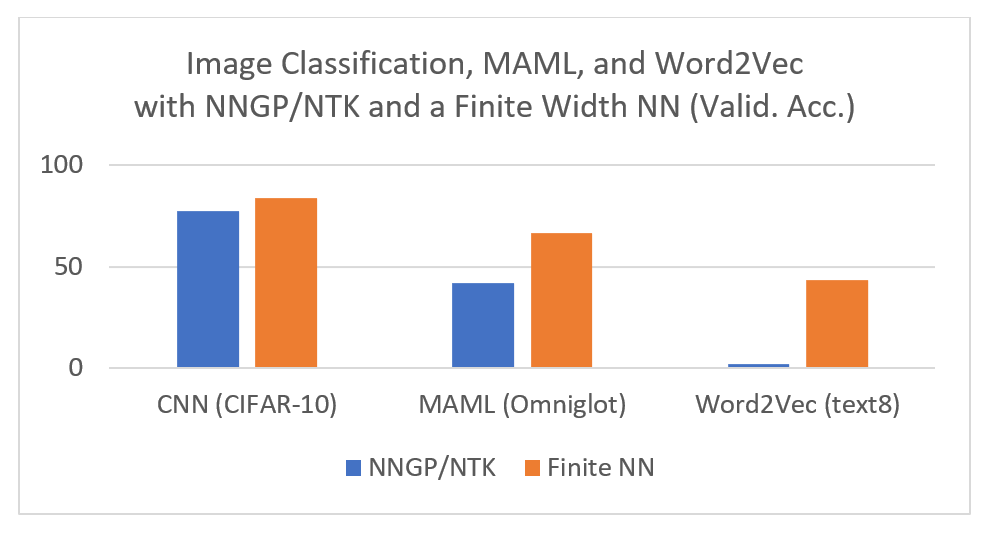Figure 1: NNGP and NTK underperform finite-width NNs on Image Classification, Word2Vec and Omniglot, even when calculating their infinite-width limits exactly. This suggests that NNGP and NTK do not capture the learning that happens in a practical NN — that is, they are not the true limit to which finite-width NNs converge. CNN result taken from Arora et al. (2019).

# Unlocking Feature Learning by Going Beyond Model Initialization

Why do NNGP and NTK fail to learn features? Because to do so, we need to leave the “comfort zone” of model initialization, where the activation coordinates are easy to analyze as they nicely follow a Gaussian law by a central limit argument—that is, summing infinitely many roughly independent, zero-mean random variables should yield a Gaussian distribution with a known variance. Just like growing a plant entails not only planting a seed but also proper care throughout its lifetime, the right infinite-width limit should take into consideration both the model initialization and the gradient updates, especially far away from initialization. To unlock feature learning, we need to see gradient updates for what they really are: a different kind of matrices from their randomly initialized counterparts.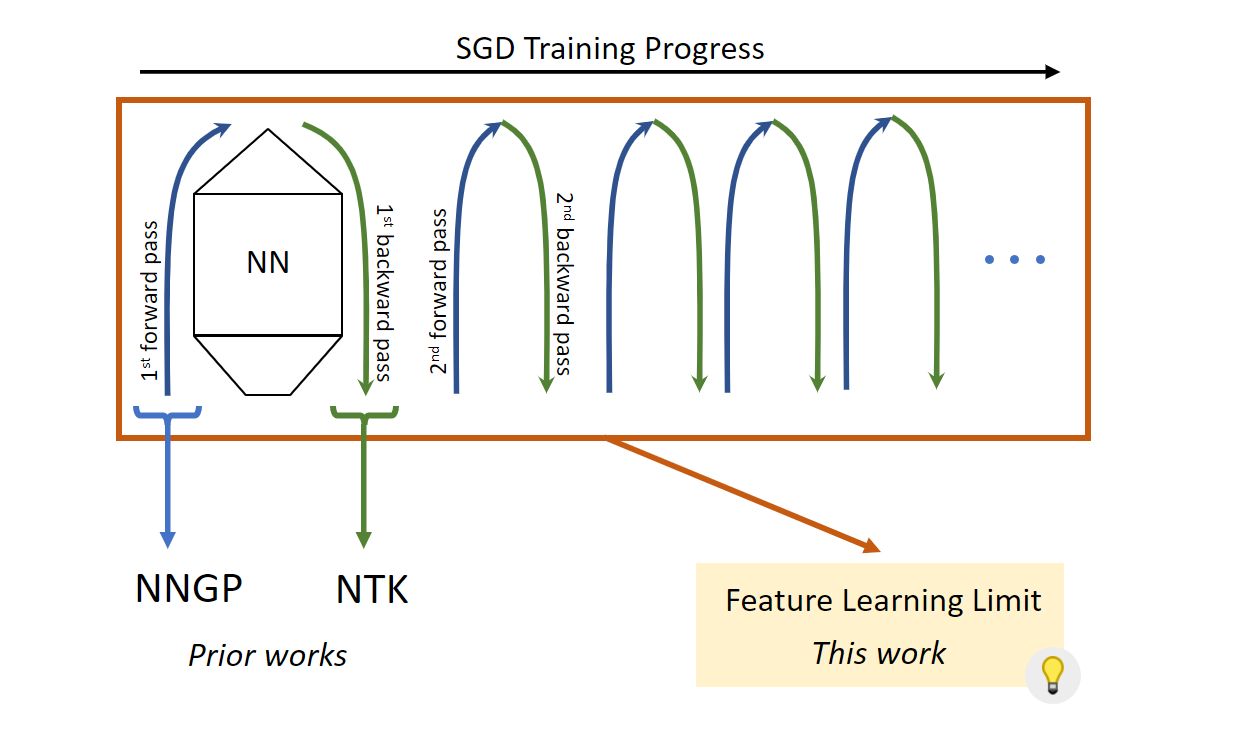Figure 2: NNGP is essentially the limit of the first forward pass in the training process, and NTK is the first backward pass. Neither leaves the “comfort zone” of model initialization and thus fails to capture feature learning. Our new limit takes into consideration the entire training process, which makes feature learning possible.

When a matrix $W \in \mathbb{R}^{n\times n}$ multiplies with an activation vector $x \in \mathbb{R}^n$ to produce a pre-activation vector, we calculate a coordinate by taking a row from the matrix $W$, multiplying it by $x$ coordinate-wise, and summing the coordinates of the resulting vector. When $W$’s entries are initialized with zero mean, this summation is across roughly independent elements with zero mean. As such, this sum would be $\sqrt{n}$ smaller than what it would be if the elements had nonzero mean or were strongly correlated, due to the famous square root cancellation effect underlying phenomena like the Central Limit Theorem.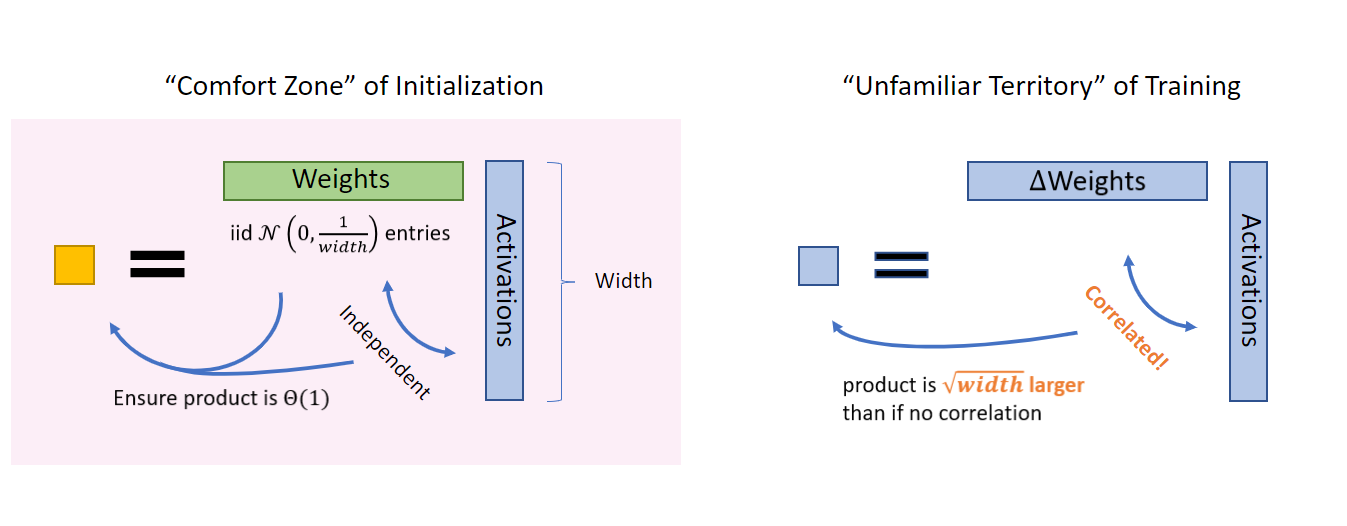Figure 3: At initialization, the weights are independent from the incoming activations, so their product is easy to reason about (for example, by using Central Limit Theorem); hence, initialization is a “comfort zone.” However, once training starts, the weights (more precisely, the change in weights, ΔWeights, due to the gradient updates) start to correlate with the activations, so we must exit this comfort zone. A Law-of-Large-Number intuition would suggest that their product is $\sqrt{width}$ larger than if there are no correlation.

In fact, this strong correlation occurs after gradient updates to $W$. Let’s focus on the gradient updates themselves, denoted as $\Delta W$. In general, the coordinates of the vector obtained by coordinate-wise multiplying a row from $\Delta W$ and the activation vector $x$ will not have zero mean. This comes partly from the fact that $\Delta W$ “remembers” the data distribution that produces the activations and partly from the model architecture (for example, the use of nonlinearity). Consequently, each entry of $\Delta W x$ will be $\sqrt{n}$ larger than if one naively assumes independence and zero-mean like at initialization.

The key to finding an infinite-width limit that admits feature learning is to carefully analyze when we have sufficient independence and zero mean and when we do not, just like our reasoning above. Now there is just one more step before we can derive such a limit.

# Not All Parameters Are the Same

Conventionally, say in a multi-layer perceptron (MLP), we treat all the parameters the same way by using the same initialization, like a Gaussian distribution with a variance of $\frac{1}{fan\_in}$, and the same learning rate. In the infinite-width limit, there are two kinds of parameters with very different behaviors—vector-like parameters and matrix-like parameters.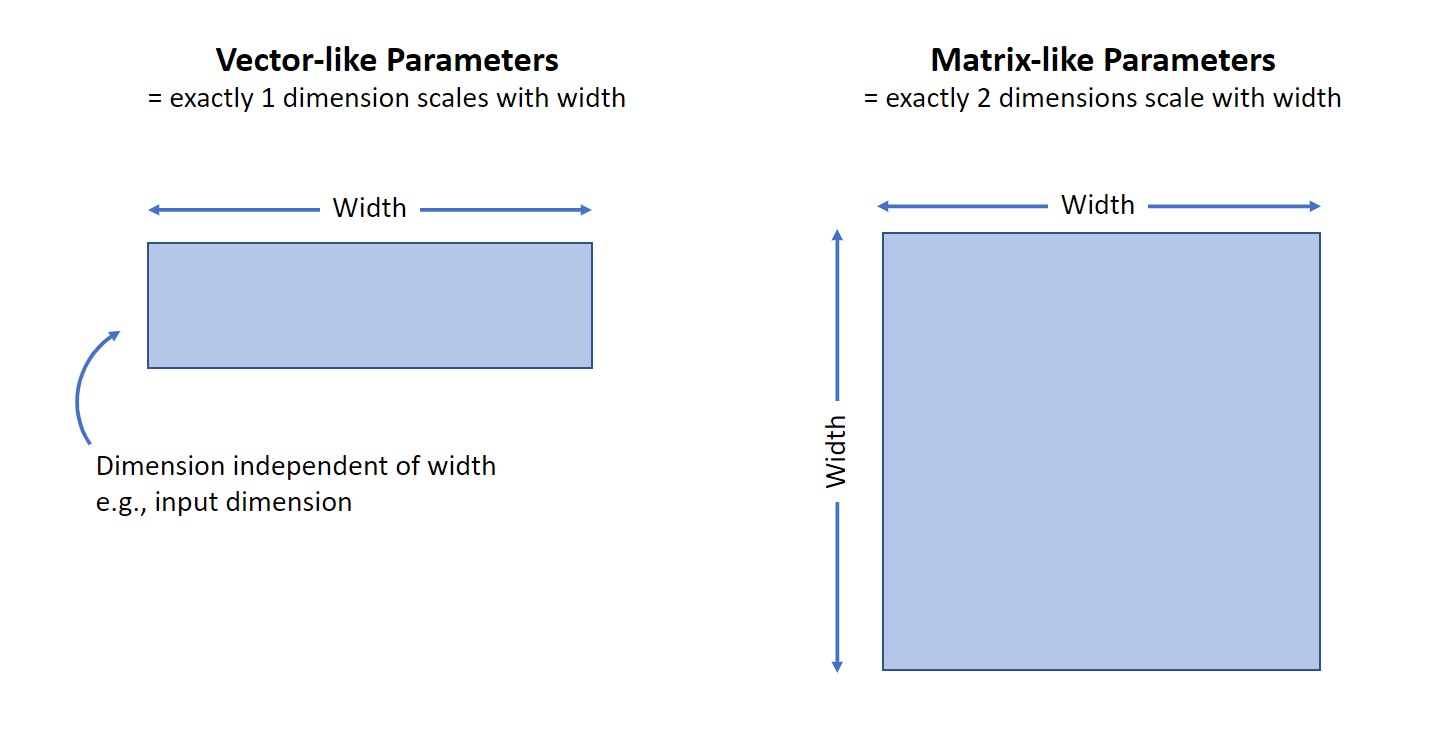Figure 4: When width is large, two kinds of parameters have different behaviors. Vector-like parameters have exactly 1 dimension scaling with width, while matrix-like parameters have exactly 2 such dimensions.

Vector-like parameters are those with exactly one dimension that scales with width — input or output layer weights and layer biases, for example. Meanwhile, matrix-like parameters have exactly two such dimensions, like hidden layer weights. The key difference is that a matrix multiplication with a vector-like parameter sometimes only sums across the finite, non-width dimension, whereas a matrix multiplication with a matrix-like parameter always sums across the width dimension, which tends to infinity. This distinction is critical in the infinite-width limit — summing infinitely many elements of size $\Theta(1)$ in width produces infinity, while summing finitely many elements each of size $\Theta(1/width)$ produces zero in the limit.

So far, we have introduced two kinds of weights: the random initialization and the gradient updates. We have also introduced two kinds of parameters: the vector-like ones and matrix-like ones. The key is to make sure that all four combinations of these lead the activations to evolve by non-vanishing and non-exploding amounts during training. Maximal Update Parametrization (abbreviated μP) scales the initialization and parameter multipliers as a function of width to ensure it for all activation vectors, thus achieving maximal feature learning. Depending on the model architecture and optimizer used, the actual parametrization could vary in complexity (see abc-parametrization in our paper). However, the underlying principles stay the same.

# Practical Impact and Looking Forward

Maximal Update Parametrization (abbreviated μP), which follows the principles we discussed and learns features maximally in the infinite-width limit, has the potential to change the way we train neural networks. For example, we calculated the limit of Word2Vec and found it outperformed both the NTK and NNGP limits as well as finite-width networks. When we visualize the learned embeddings of two groups of words — the names of American cities and those of states — using Principal Component Analysis, we see that μP’s limit exhibits a clear separation between them, like in the finite neural network, while the NTK/NNGP limit sees essentially random embeddings.

“The theory of wide feature learning is extremely exciting and has the potential to change the way the field thinks about large model training.”

Ilya Sutskever, Co-founder and Chief Scientist at OpenAI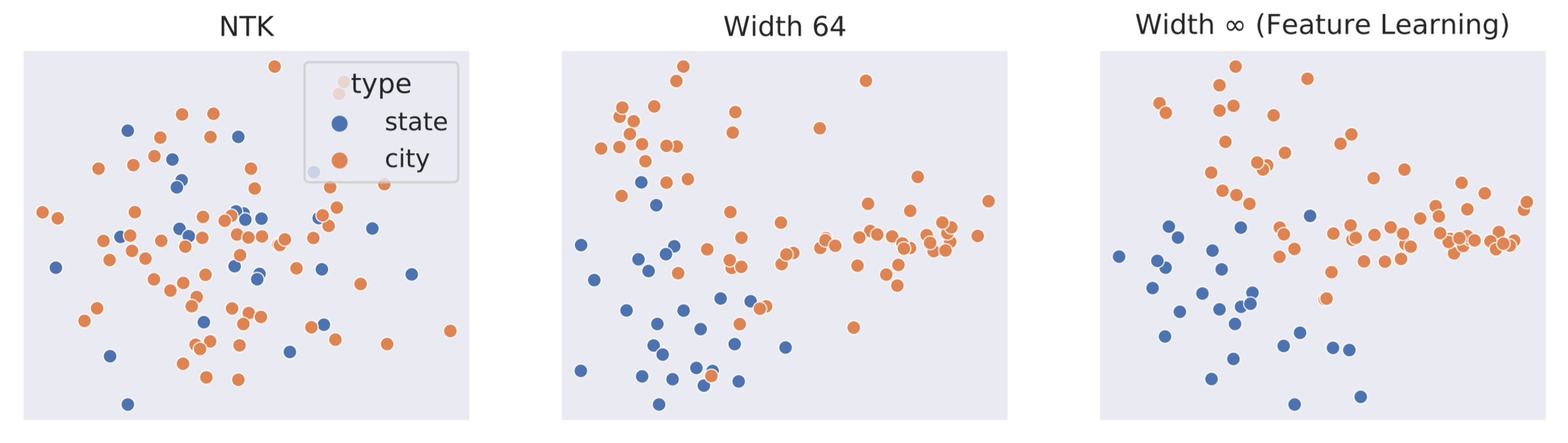Figure 5: Principal Component Analysis of Word2Vec embeddings of common US cities and states, for NTK, width-64, and width-∞ (feature learning) neural networks. NTK embeddings (left plot) are essentially random — you can see that there is no separation of cities and states in the far left embeddings above. In contrast, cities and states get naturally separated in the embedding space as width increases in the feature learning regime. In the width-64 model (middle plot), some separation can be seen, and even more separation can be seen in the infinite-width model (right plot).

Parametrizing a model in allows it to retain the ability to learn features when its width goes to infinity — that is, the model does not become trivial (like NTK and NNGP) or run into numerical issues in the limit. We believe this new perspective opens doors to new capabilities previously unimaginable. Indeed, our theory enables a novel and useful paradigm for training large models, such as GPT and BERT, which is the topic of one of our on-going projects. Our results also raise several questions about existing practices, for example, about uncertainty in Bayesian neural networks. “These results are also intriguing because they suggest that the infinite width-limit of feature learning leads to a deterministic training trajectory and thus precludes the use of variance due to initialization to ascertain model uncertainty,” Yoshua Bengio explains. “This should inspire future works on better uncertainty estimation in the feature learning regime.”

Due to the dominance of Neural Tangent Kernel theory, many researchers in the community believed that large width causes neural networks to lose the ability to learn features. We decisively refute this belief in our work. However, rather than an end to a chapter, we believe this is just a new beginning with many exciting new possibilities. We welcome everyone to join us on this journey to unveil the mysteries of neural networks and to push deep learning to new heights.

• Read our paper for a deeper dive into the technical aspects.
• Discover more about feature learning and infinite-width networks in a presentation by Greg Yang.
• Train your own infinite-width feature learning neural network with our GitHub repository.
• Discover questions and comments from the machine learning community on this Reddit thread.
]]>
Randomized Smoothing of All Shapes and Sizes, Part I2020-05-06T14:00:00+00:002020-05-06T14:00:00+00:00https://decentdescent.org/rs4a1

Can we find a general theory for randomized smoothing? We show that for an appropriate notion of “optimal”, the best smoothing distribution for any norm has level sets given by the Wulff Crystal of that norm.

In the previous post, we discussed how to learn provably robust image classifiers via randomized smoothing and adversarial training, robust against $\ell_2$ adversarial perturbations. There, the choice of Gaussian for the smoothing distribution would only seem most natural. What if the adversary is $\ell_p$ for $p \ne 2$? What kind of distributions should we smooth our classifier with? In this post, we take a stroll through a result of our recent preprint Randomized Smoothing of All Shapes and Sizes that answers this question, which curiously leads us to the concept of Wulff Crystals, a crystal shape studied in physics for more than a century. Empirically, we use this discovery to achieve state-of-the-art in $\ell_1$ provable robustness in CIFAR10 and Imagenet.

# Introduction

While neural networks have excelled at image classification tasks, they are vulnerable to adversarial examples; imperceptible perturbations of the input that change the predicted class label (Szegedy et al. 2014), as illustrated by the following image from Madry and Schmidt’s blog post.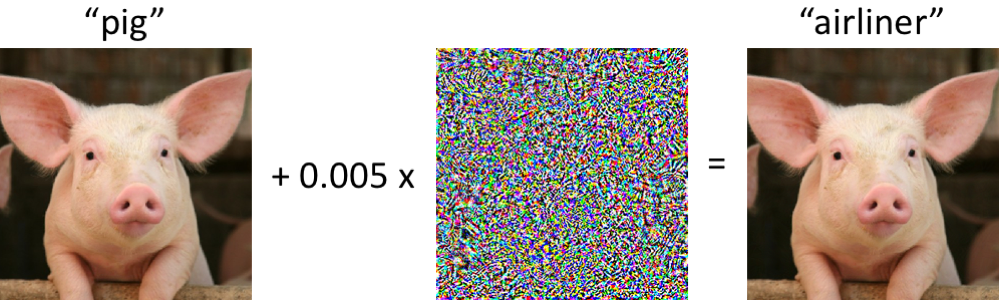This has most often been formalized in terms of the following desiderata: Let $\mathcal{B}$ be a set of “allowed adversarial perturbations,” such as $\mathcal{B} = \{v \in \mathbb{R}^d: \|v\|_\infty\leq 8/255\}$. Consider a classifier $f$ that maps an input $x$ (e.g. an image) to one of a number of classes (e.g. the label “cat”). Then we say a function $f$ is robust at $x$ to perturbations from $\mathcal B$ if $f(x)$ and $f(x+v)$ share the same labels for all $v \in \mathcal B$.

It is easy to create such inputs by perturbing a data point $x$ by, for example, projected gradient descent (Madry et al. 2018) (see our previous post for a concise overview). This raises important safety concerns as machine learning is increasingly deployed in contexts such as healthcare and autonomy.

The community has developed a plethora of techniques toward mending a model’s vulnerability to adversarial examples: adversarial training, gradient masking, discretizing the input, etc. Yet these “empirical defenses” are usually short-lived and completely broken by newer, more powerful attacks (Athalye et al. 2018).

This motivates the study of certifiably robust defenses, which have emerged to stop this escalating arm race. In such a defense, the classifier not only labels the input, but also yields a certificate that says “not matter how the input is perturbed by a vector in $\mathcal B$, my label will not change.”

We focus on randomized smoothing, a method popularized by Lecuyer et al. 2018, Li et al. 2018, and Cohen et al. 2019 that attains state-of-the-art certifiable robustness guarantees. The core idea is simple: given a classifier $f$ and input $x$, instead of feeding $x$ directly to $f$, we can sample lots of random noise, say from a normal distribution, $(\delta^{(1)},\dots,\delta^{(m)}) \sim N(0, \sigma^2 I)$, and output the most commonly predicted class among the predictions $f(x+\delta^{(1)}),\dots,f(x+\delta^{(m)})$. It turns out that this scheme can guarantee this most common class stays unchanged when the input $x$ is perturbed by vectors of small $\ell_2$ norm. We will more formally discuss this technique below. For more background, we also welcome the reader to see our previous post.

For the most part, prior works on randomized smoothing has focused on smoothing with a Gaussian distribution and defending against $\ell_2$ adversary, neglecting other traditionally important threat models like $\ell_\infty$ or $\ell_1$. Here, we take a step back and ask the following:

What’s the optimal smoothing distribution for a given $\ell_p$ norm?

In answering, we will achieve state-of-the-art empirical results for $\ell_1$ robustness on CIFAR-10 and ImageNet datasets. In the table below we show the certified accuracies comparing our choice of smoothing with the Uniform distribution to the previous state-of-the-art which performed smoothing with the Laplace distribution. Here, each percentage indicates the fraction of the test set for which we both 1) classify correctly and 2) guarantee there is no adversarial example within the corresponding $\ell_1$ radius.

ImageNet $\ell_1$ Radius 0.5 1.0 1.5 2.0 2.5 3.0 3.5 4.0
Uniform, Ours (%) 55 49 46 42 37 33 28 25
Laplace, Teng et al. (2019) (%) 48 40 31 26 22 19 17 14
CIFAR-10 $\ell_1$ Radius 0.5 1.0 1.5 2.0 2.5 3.0 3.5 4.0
Uniform, Ours (%) 70 59 51 43 33 27 22 18
Laplace, Teng et al. (2019) (%) 61 39 24 16 11 7 4 3

# Background

Let us first introduce some background on randomized smoothing.

Definition. Let $\mathcal Y$ be a finite set of labels (e.g. “cats”, “dogs”, etc). Let $f:\mathbb{R}^d\mapsto \mathcal{Y}$ be a classifier that outputs one of these labels given an input. Given a distribution $q$ on $\mathbb R^d$, we can smooth $f$ with $q$ to produce a new classifier $g$, called the smoothed classifier, which is defined at any point $x \in \mathbb R^d$ as

$g(x) = \underset{c \in \mathcal{Y}}{\arg \max}\ \underset{\delta \sim q}{\mathrm{Pr}} [f(x + \delta)=c].$

A moment of thought should show that $g$ is the same classifier as the one produced by the “majority vote” procedure for randomized smoothing described in the introduction, if we have an infinite number of random noises $\delta^{(1)}, \delta^{(2)}, \ldots \sim q$.

Slightly less intuitively, we can also define $g(x)$ in the following way, in terms of the decision regions of the base classifier $U_c = \{ x' \in \mathbb{R}^d : f(x') = c\}$:

$g(x) = \underset{c \in \mathcal{Y}}{\arg\max}\ q(U_c - x).$

Here $q(U) = \mathrm{Pr}_{\delta\sim q}[\delta \in U]$ and $U_c-x$ denotes the translation of $U_c$ by $-x$. Note $q(U_c - x)$ is the same as the measure of $U_c$ under the recentered distribution of $q$ at $x$, and $g(x)$ is the maximum such measure over all decision regions $U_c, c \in\mathcal Y$.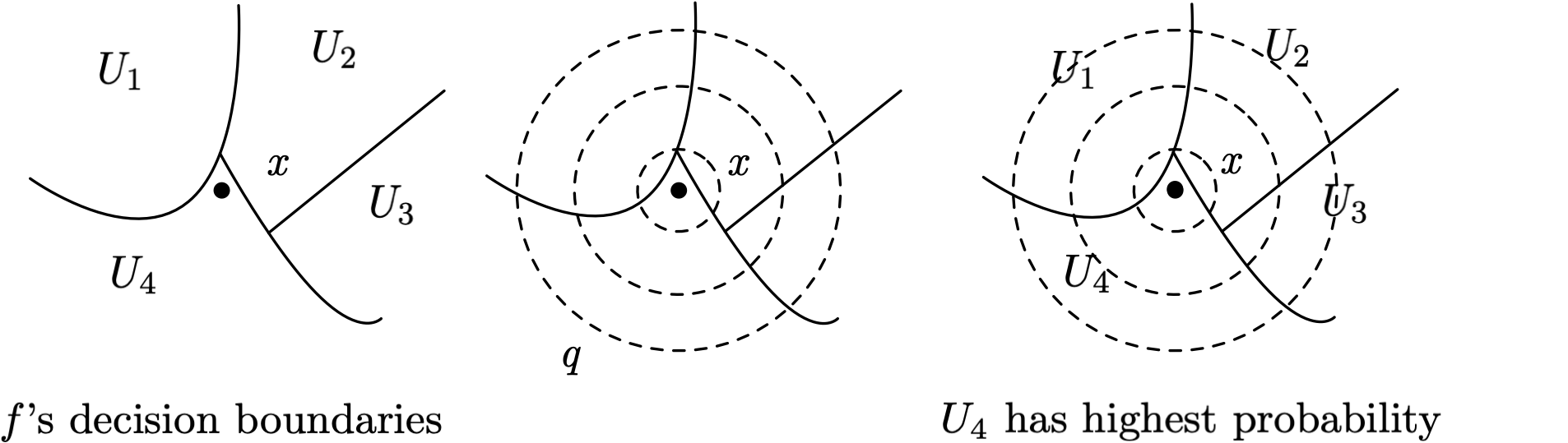Now let’s turn our attention to what robustness properties arise in $g(x)$, the smoothed classifier. To do so, we’ll need to consider the worst case over all possible decision regions $U_c$ that may arise, since the base classifier is an arbitrary neural network.

Definition. The growth function $\mathcal G$ with respect to distribution $q$ is defined as,

$\mathcal{G}_q(p, v) = \sup_{U\subseteq \mathbb{R}^d: q(U)=p}q(U-v), \text{for any } p \in[0, 1], v \in \mathbb{R}^d$

Its interpretation is: out of all sets $U$ (i.e. all possible decision regions) with initial measure $p$, what is the most that its measure can increase when we shift the center of $q$ by a vector $v$? Note this is a property of $q$ alone and does not depend on $x$.

For example, consider a 2D Gaussian measure $q = N(0,I)$ with a perturbation $v=[1,1]$. Let $p=\frac{1}{4}$ and consider two possible choices: let $U_1$ be the bottom-left quadrant and $U_2$ be the top-right quadrant. They both have measure $p=\frac 1 4$ under $q$. It’s easy to see that after a shift in the distribution’s center by $v$, $U_2$ will have higher measure than $U_1$.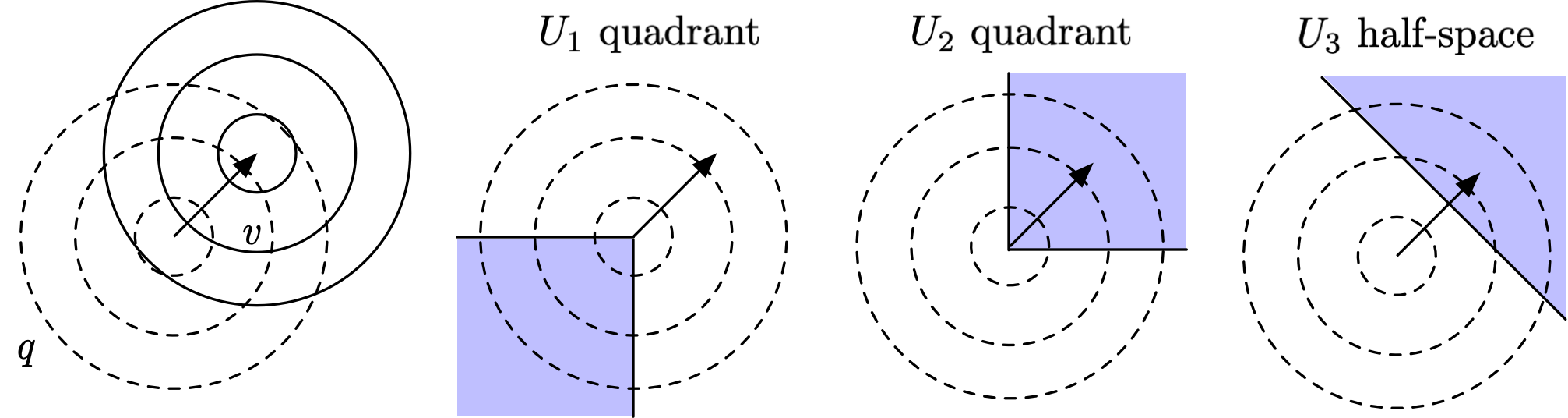It turns out that here the most extreme choice of $U$ with measure $p=\frac{1}{4}$, in terms of maximizing the growth function, is $U_3$, the half-space formed with a hyper-plane perpendicular to $v$, with boundary positioned such that $q(U_3)=\frac{1}{4}$.

To see this, think of the problem of finding the most extreme $U$ as a problem of “maximizing value under budget constraint.” Each vector $u$ added to $U$ will “cost” $q(u)$, and the total cost is at most $p$, the measure of $U$ under $q$. At the same time, each $u$ will also bring in a “profit” of $q(u-v)$, and the total profit is $q(U-v)$, the measure of $U$ under $q(\cdot -v)$. Thus a greedy cost-benefit analysis would suggest that we sort all $u \in \mathbb R^d$ by $\frac{q(u -v)}{q(u)}$ in descending order, and add to $U$ the top $u$s until we hit our cost budget, i.e. $\int_{U} q(u)du = p$. In the above example of $q$ being Gaussian, the ratio $\frac{q(u -v)}{q(u)}$ changes only along the direction $v$ and stays constant in each hyperplane perpendicular to $v$, so the above reasoning confirms that the half-space $U_3$ above is the maximizing $U$. The growth function in this example would then read $\mathcal G_q(\frac 1 4, v) = q(U_3-v)$, which turns out to have a simple expression that we shall describe below.

If the smoothed classifier $g$ assigns label $c$ to input $x$, then $U_c - x$ has the largest measure under $q$ among all decision regions $\{U_c: c \in \mathcal Y\}$. Thus, $g(x+v) = g(x)$ if $U_c-x$ does not shrink in $q$-measure too much when it is shifted by $-v$, or equivalently if the complement of $U_c-x$ does not grow too much in $q$-measure. This intuition can be captured more formally with the growth function:

Result. Suppose for a base classifier $f$, we have $\rho = \mathrm{Pr}_{\delta \sim q}[f(x+\delta) = c] > \frac{1}{2}$. Then the smoothed classifier $g$ will not change its prediction under perturbation set $\mathcal{B}$ if

$\sup_{v\in\mathcal{B}} \mathcal{G}_q(1-\rho, v)< \frac{1}{2}.$

Back to our 2D Gaussian example, and suppose we have $\rho=1-p=0.75$ and recall that we’re interested in a perturbation $v=[1,1]$. From the above result we know that $g$ will not change its prediction as long as $\mathcal{G}_q\left(1-0.75, [1,1]\right)<\frac{1}{2}$. So we need to calculate $\mathcal{G}_q\left(0.25, [1,1]\right)$, which is the measure of the half-space we identified earlier, under the distribution $q$ shifted by $[1,1]$. Where is the boundary of the half-space? Consider a change of basis so that the x-axis follows the direction of $v$ such as in the center figure below. The boundary occurs at $x=\Phi^{-1}\left(0.75\right)$. What’s the measure of this half-space under $q$ shifted by $[1,1]$? Exactly $1-\Phi(\Phi^{-1}(\frac{3}{4}) - \sqrt{2})$.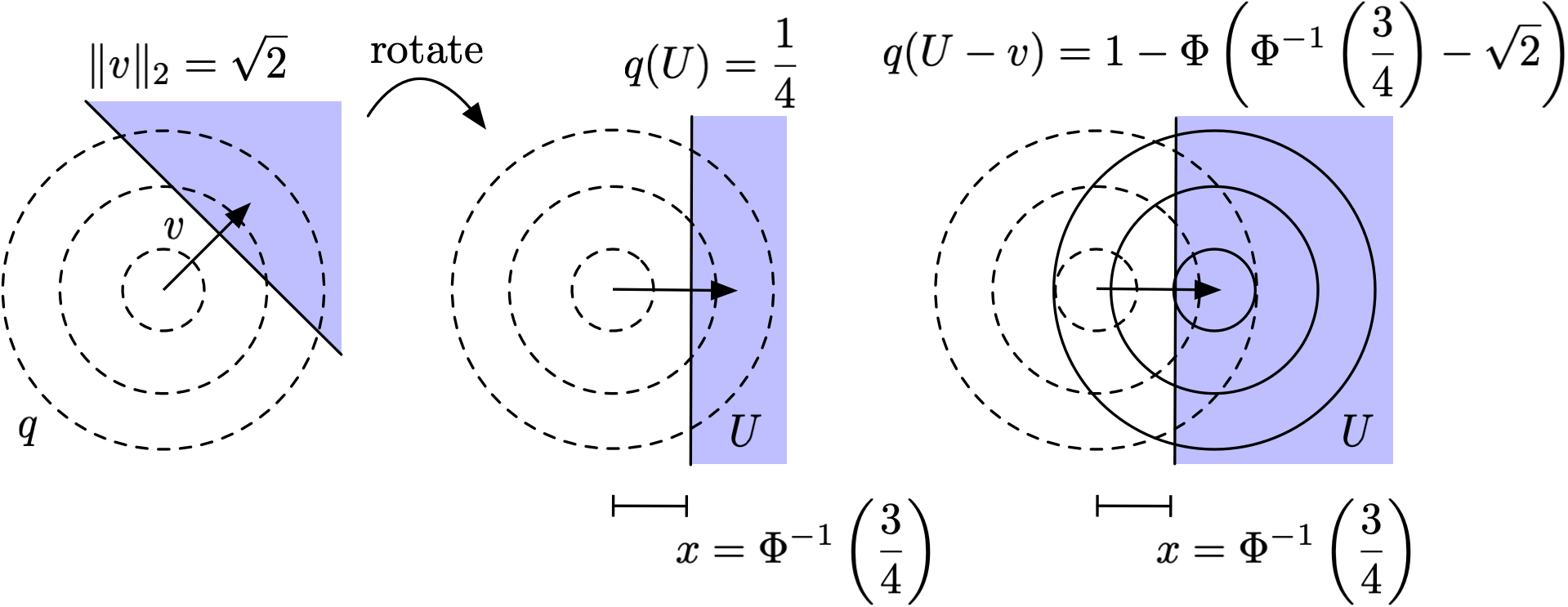Therefore,

$\mathcal{G}_q\left(0.25, [1, 1]\right)= 1-\Phi\left(\Phi^{-1}\left(0.75\right)-\sqrt 2\right) \approx 0.77 > 0.5,$

so the classifier is not robust to the perturbation if $\rho=0.75$. On the other hand, following similar logic we can calculate the growth function for $\rho=0.99$, which tells us

$\mathcal{G}_q(0.01, [1,1]) = 1 - \Phi(\Phi^{-1}(0.99)-\sqrt 2) \approx 0.41 < 0.5,$

so the classifier is robust to the perturbation if $\rho=0.99$.

More generally Gaussian smoothing yields the bound derived in Cohen et al. 2019,

$\mathcal{G}_q(1-\rho,v) = 1-\Phi\left(\Phi^{-1}(\rho) - \frac{\|v\|_2}{\sigma}\right).$

# The Optimal Smoothing Distributions

Here we provide intuition for the question we want to answer:

What is the best distribution to smooth with if we want $\ell_p$ robustness?

First let’s simplify and consider a uniform distribution over a convex set $S\subseteq \mathbb{R}^d$ where $\mathrm{Vol}(S)=1$. By inspection,

$\mathcal{G}_q(p,v)=\min(1, p + \mathrm{Vol}((S + v) \setminus S)).$

In this situation, the $U$ that obtains the $\sup$ for the growth function is any subset of $(S + v )\cap S$ with volume $p$, unioned with the complement of $S$. For example, see the left figure below, in which the area of $U \cap S$ is $p$, satisfying $q(U)=p$ where $q$ is the measure of our uniform distribution.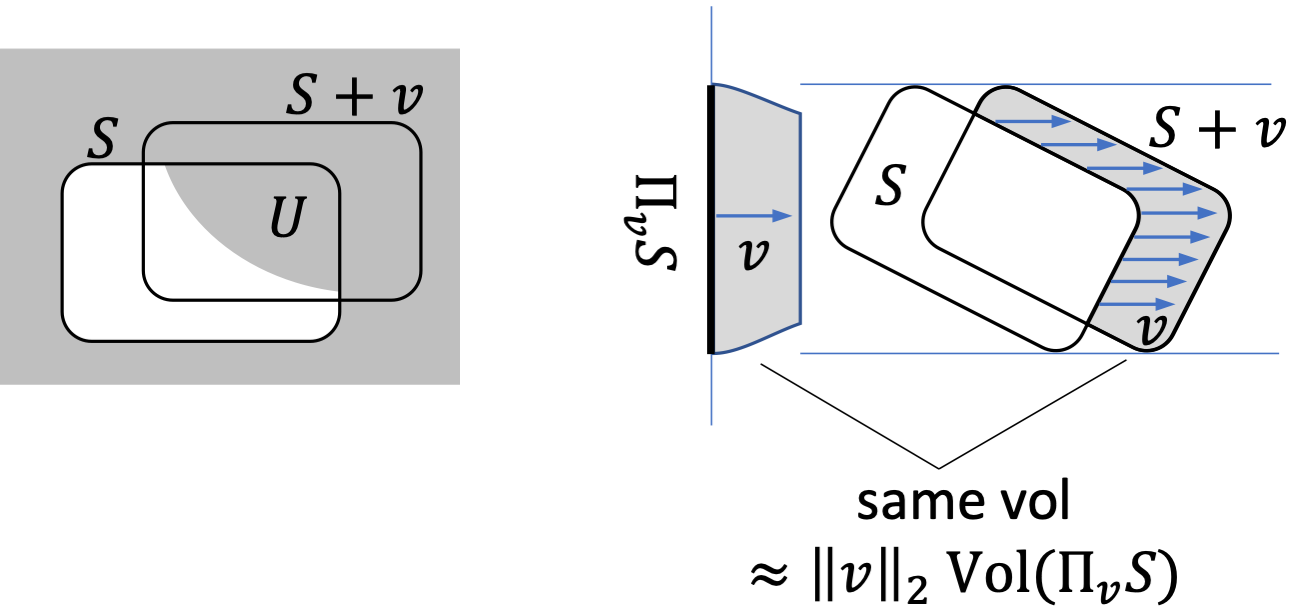Now consider an infinitesimal translation by $rv$. As $r\rightarrow 0$, the volume of $(S + v) \setminus S$ approaches $r$ times the volume of the projection $\Pi_v S$ of $S$ along $v$, in terms of $(d-1)$-dimensional Lesbegue measure. Here, $\Pi_v S$ is formally defined as $\{u - \frac{u^\top v}{\|v\|^2} v: u \in S\}$. See the right figure above for an example in 2D – the projection $\Pi_v S$ of $S$ along $v$ yields the length between the two blue horizontal lines.

This intuition justifies the following limits:

$\lim_{r\rightarrow 0} \frac{\mathcal{G}_q(p, rv) - p}{r} = \lim_{r\rightarrow 0}\frac{\mathrm{Vol}((S+rv)\setminus S)}{r}= \|v\|_2\mathrm{Vol}(\Pi_v S).$

In the context of randomized smoothing, recall that the smoothed classifier is robust to a perturbation $v$ as long as $\mathcal{G}_q(1-\rho,v)<\frac{1}{2}$. Here in our uniform distribution example, this means that $g$ is robust to small perturbations $rv\in r\mathcal{B}$ as long as

\begin{aligned} \mathcal{G}_q(1-\rho, rv) & \approx 1-\rho + r\| v\| _2 \mathrm{Vol}(\Pi_v S) < \frac{1}{2}\\ \iff & r < \frac{\frac{1}{2} - (1-\rho)}{\|v\|_2\mathrm{Vol}(\Pi_vS)}. \end{aligned}

If we consider the worst-case perturbation $rv\in r \mathcal{B}$ for a fixed model, we have a smoothed classifier robust to a perturbation set $\mathcal{B}$ as long as

$\sup_{rv\in r\mathcal{B}}\mathcal{G}_q(1-\rho,rv) \approx 1-\rho + r\sup_{v\in\mathcal{B}}\|v\|_2\mathrm{Vol}(\Pi_v S) <\frac{1}{2}.$

Importantly, the model is more robust as $\sup_{v\in\mathcal{B}}\|v\|_2 \mathrm{Vol}(\Pi_v S)$ decreases. So a natural question to ask is, which set $S$, our smoothing distribution, minimizes this quantity over all convex sets of volume $1$?

It turns out the answer is the Wulff Crystal, a shape from theoretical physics.

# Wulff Crystals

Definition. The Wulff Crystal with respect to $\mathcal{B}$ is defined as the unit ball of the norm dual to $\|\cdot\|_\ast$, where $\|x\|_\ast= \mathbb{E}_{y\sim\mathrm{Vertices}(\mathcal{B})} \vert x^\top y \vert$ with $y$ sampled uniformly from vertices of $\mathcal{B}$.

Let’s unpack this a bit. Recall dual norm is defined as,

$\|z\| = \sup \{ z^\top x : \|x\|_\ast \leq 1\}.$

For example, it’s well known that the dual norm of the $\ell_p$ norm is the $\ell_q$ norm where $\frac{1}{p} + \frac{1}{q} = 1$.

Now suppose we’re interested in the Wulff Crystal for $\mathcal{B}$, where $\mathcal{B}$ is the $\ell_1$ rhombus in two dimensions, which has vertices $\{ (0, 1), (0, -1), (1, 0), (-1, 0)\}$. Thus,

\begin{aligned} \mathbb{E}_{y\sim \mathrm{Vertices}(\mathcal{B})}|x^\top y| & = \frac{1}{2}|x_1| + \frac{1}{2}|x_2| \end{aligned}

So the constraint $\|x\|_\ast \leq 1$ corresponds to constraining $\|x\|_1 \leq 2$ i.e. a scaled version of the $\ell_1$ rhombus. What’s the dual of this? For any $z$, $\sup\{z^\top x:\|x\|_1 \leq 2\}=2\|z\|_\infty$, so the Wulff Crystal is the ball $\|z\|_\infty\leq \frac{1}{2}$.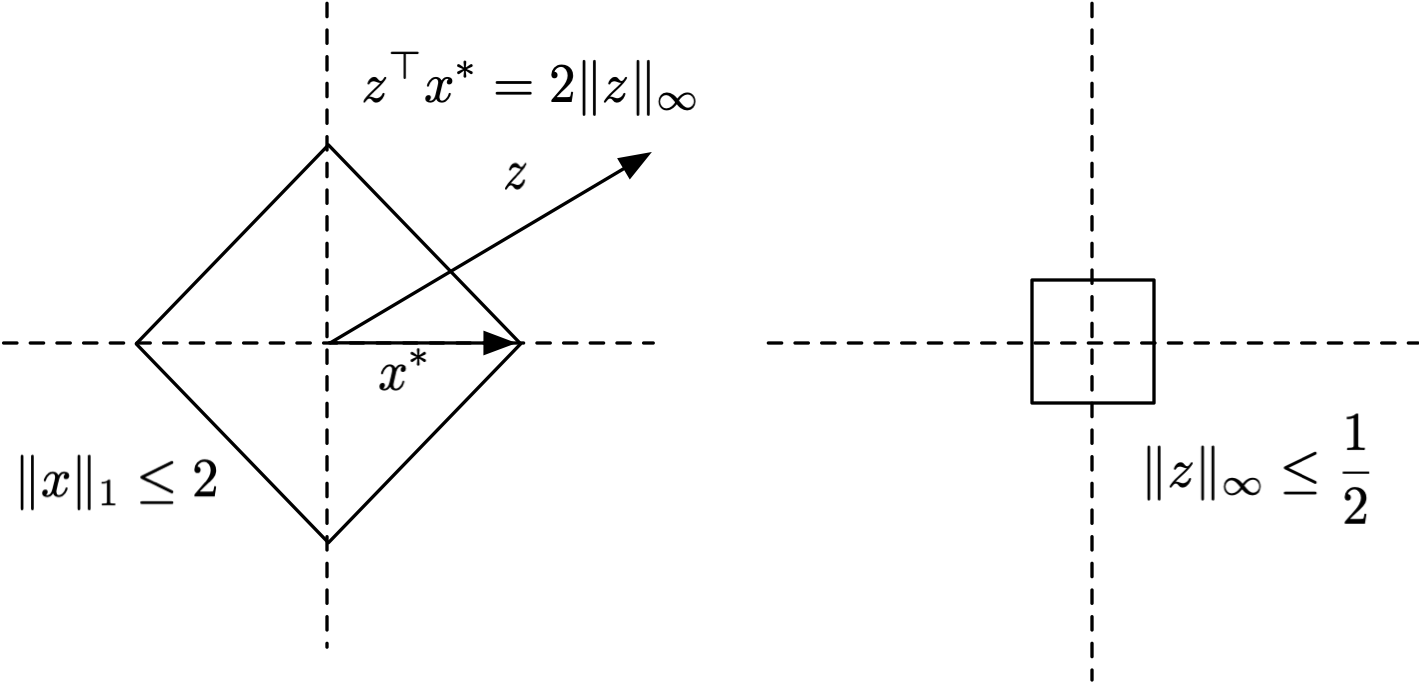Below we show a few examples of Wulff Crystals for different perturbation sets $\mathcal{B}$.

• For the $\ell_1$ cross-polytope, the Wulff Crystal is a cube.
• For the $\ell_2$ sphere, the Wulff Crystal is a sphere.
• For the $\ell_\infty$ cube, the Wulff Crystal is the zonotope (i.e. Minkowski sum) of vectors $\{\pm 1\}^d$. In two dimensions this is a rhombus and in three dimensions it is a rhombic dodecahedron, but in higher dimensions there is no simpler description of it than as the zonotope of the vectors $\{\pm 1\}^d$.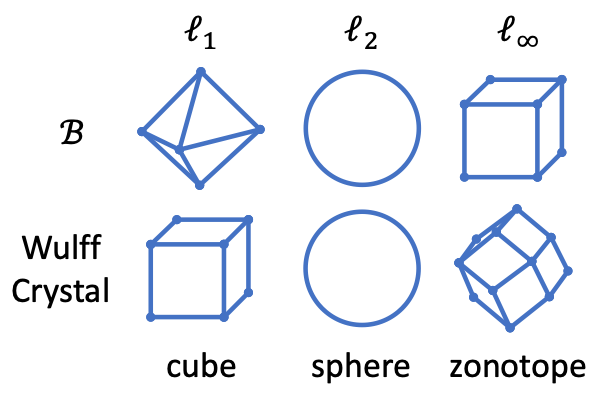Result. For any $p \in [0, 1)$, the Wulff Crystal with respect to $\mathcal{B}$ minimizes the quantity

$\sup_{v\in\mathcal{B}}\lim_{r\rightarrow 0} \frac{\mathcal{G}_q(p, rv) - p}{r} = \sup_{v\in\mathcal{B}}\lim_{r\rightarrow 0} \frac{1}{r}\mathrm{Vol}((S + rv) \setminus S)$

among all measurable (not necessarily convex) sets $S$ of the same volume, and when $\mathcal{B}$ is sufficiently symmetric (e.g. $\ell_1,\ell_2,\ell_\infty$ balls).

In other words, setting $S$ to be the Wulff Crystal minimizes the growth function, and therefore makes $g$ more robust. So this covers cases of smoothing with a distribution that’s uniform over a set $S$. What about the more general (i.e. non-uniform) case? It turns out the Wulff Crystal remains optimal, in that it determines the best shape of the distribution’s level sets.

Result. Let $q_0$ be any reasonable distribution with an even density function. Among all reasonable and even density functions $q$ (i.e. $q(x) = q(-x)$) with superlevel sets $\{x: q(x) \geq t\}$ with the same volumes as those of $q_0$, the quantity

$\sup_{v\in\mathcal{B}}\sup_{q(U)=\frac{1}{2}} \lim_{r\rightarrow 0 } \frac{q(U-rv)-\frac{1}{2}}{r}$

is minimized by the distribution $q^\ast$ with superlevel sets proportional to the Wulff Crystal with respect to $\mathcal{B}$. For proof, see our paper.

This means that:

• For the $\ell_1$ ball, the distribution should have cubical level sets.
• For the $\ell_2$ ball, the distribution should have spherical level sets.
• For the $\ell_\infty$ ball, the distribution should have zonotope-shaped level sets.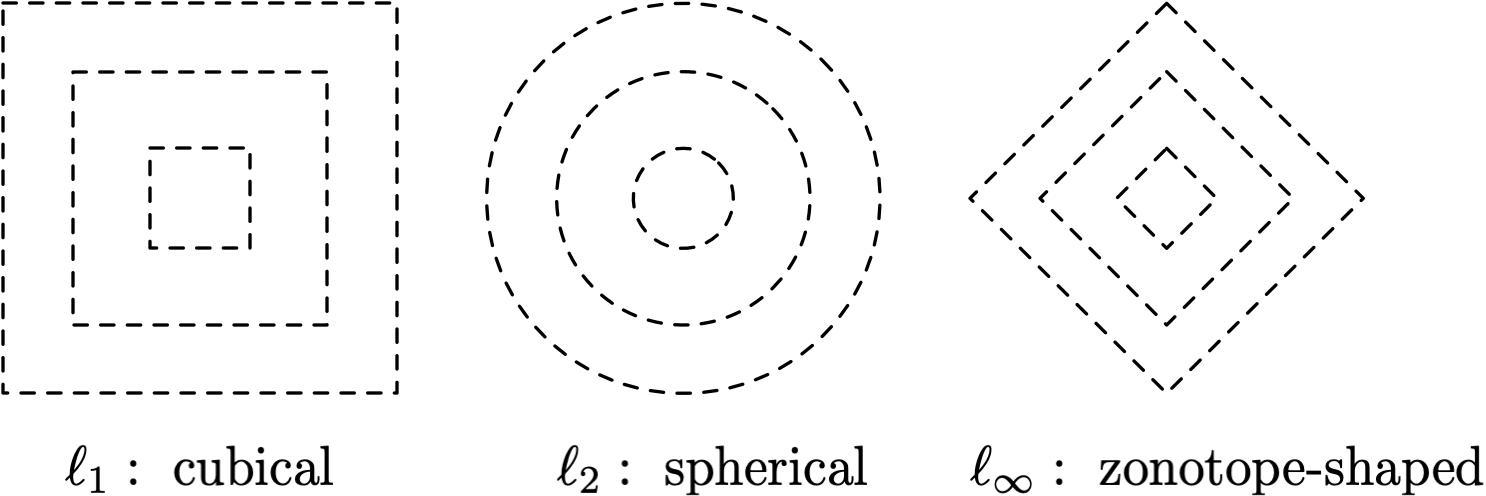We note that an important difference between this result and the previous one is that it applies specifically to the case where $q(U)=\frac{1}{2}$, not the more general case for any value of $1-\rho$. In the context of randomized smoothing, this corresponds to the case where the smoothed classifier correctly classifies the class $c$, but only barely i.e.

$\rho = \mathrm{Pr}_{\delta\sim q }[f(x+\delta)= c] = \frac{1}{2} + \epsilon.$

This is an important case, as such inputs $x$ are close to the decision boundary, and Wulff Crystal distributions yield the best robustness guarantee for such hard points.

Another way to look at this: Any choice of smoothing distribution $q$ implies a different certified radius against an $\ell_p$ adversary, given a fixed value of $\rho$. For example, below we plot the radii for the $\ell_1$ adversary and for Gaussian, Laplace, and Uniform distributions. What this theorem says is that the slope of the curve at $\rho=0.5$ will be highest for the distribution with Wulff Crystal level sets.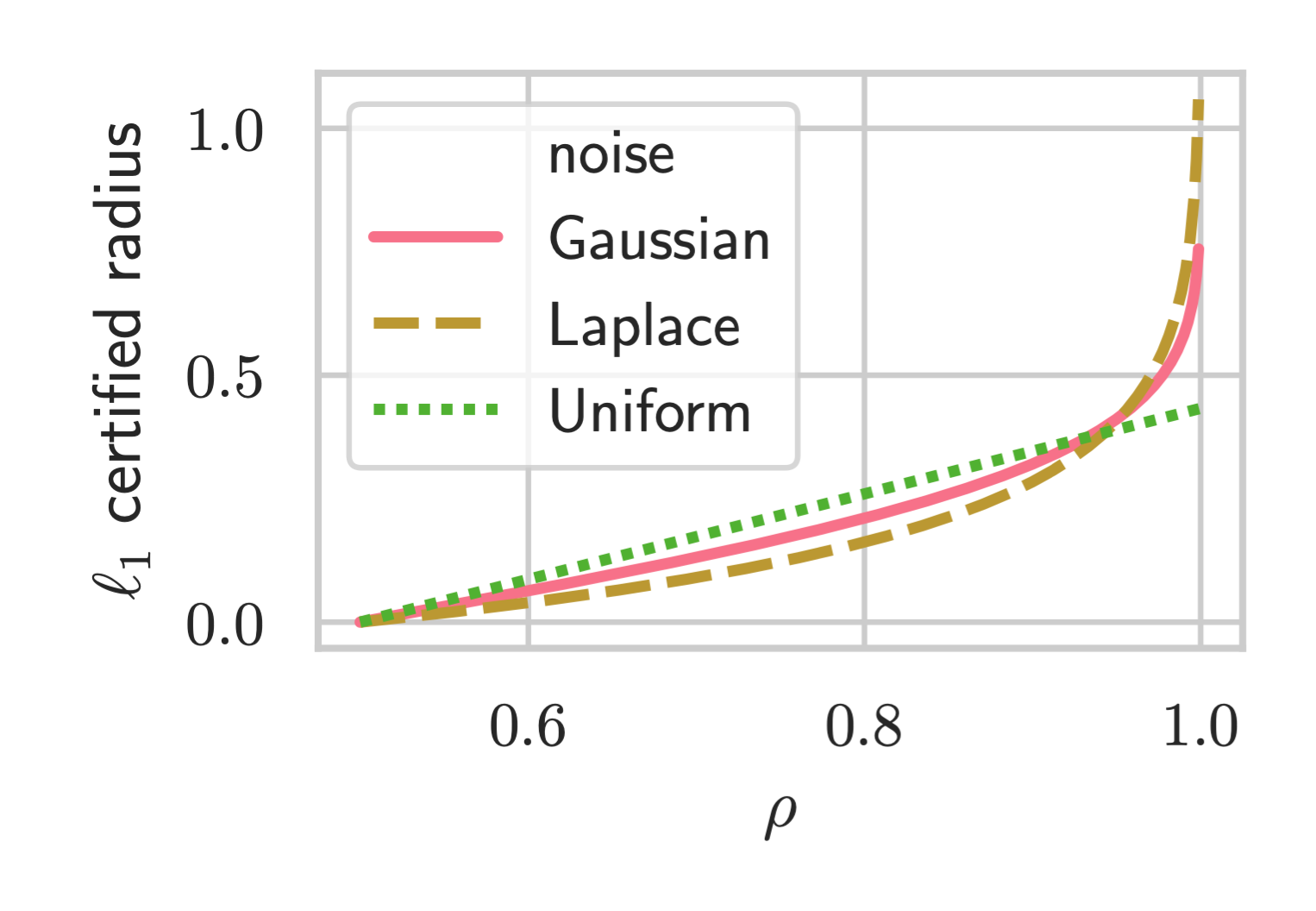# Empirical Results

We ran an extensive suite of experiments on ImageNet and CIFAR-10 to verify our Wulff Crystal theory. In this section we’ll focus on the $\ell_1$ adversary, whose Wulff Crystal is cubical. We therefore compare the following distributions:

1. Uniform (cubical), $\mathbb{I}\{\|x\|_\infty \leq \lambda\}$.
2. Gaussian (non-cubical), $\propto e^{-\|x/\lambda\|_2^2}$.
3. Laplace (non-cubical), $\propto e^{-\|x/\lambda\|_1}$.

We also compare against a larger array of other distributions in our paper, but we shall focus on just the above in this post. We use Wide ResNet for all of our experiments.

Definition. The certified robust accuracy at an $\ell_p$ radius of $\epsilon$ is the fraction of the test set the smoothed classifier $g$ correctly classifies and certifies robust for at least a ball of size $\epsilon$. Since larger noise variances $\sigma^2 = \mathbb{E}_{\delta\sim q}[\|\delta\|^2_2]$ naturally lead to larger robust radii but trade off against accuracy, we tune over the noise variance and consider the best certified robust accuracy among all values of the hyper-parameter.

Below we show the certified top-1 accuracies for CIFAR-10 and ImageNet. We find that the Uniform distribution performs best, significantly better than Gaussian and Laplace distributions.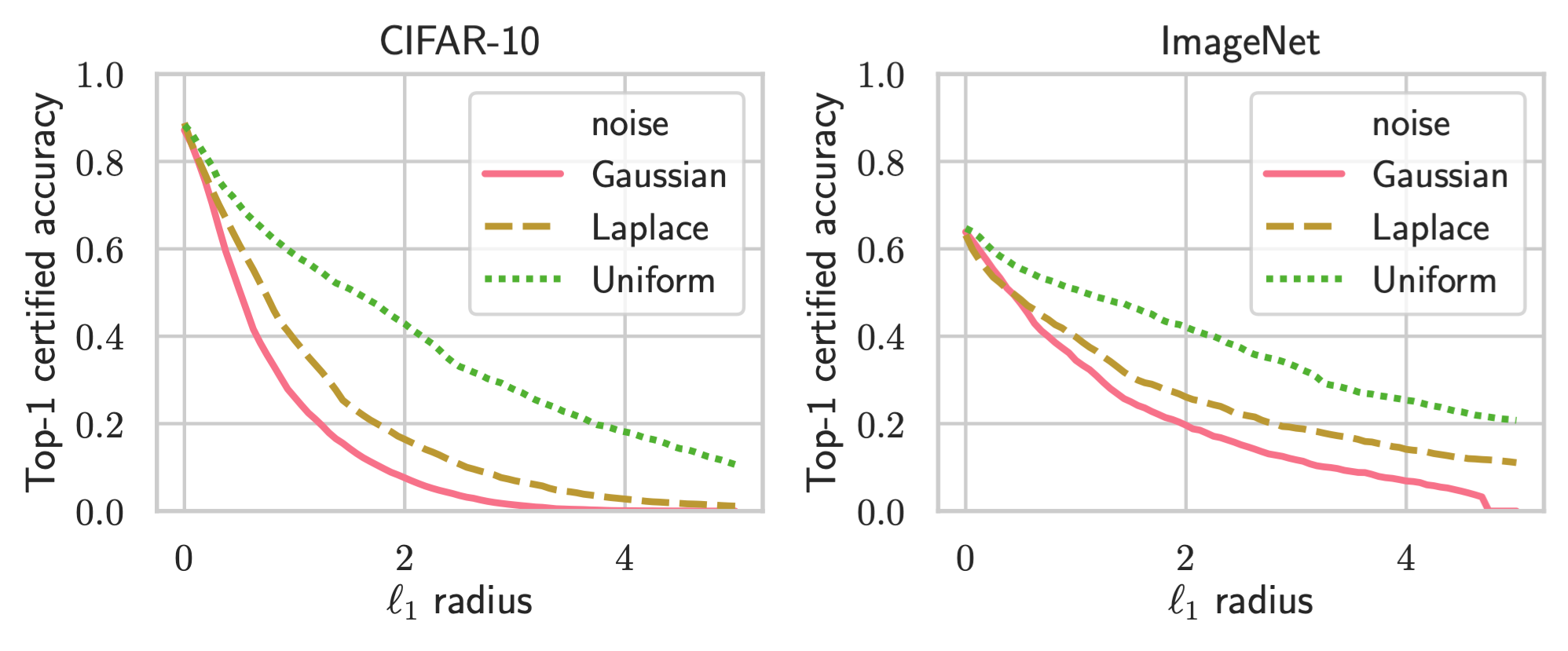# Conclusion

In this blog post, we reviewed randomized smoothing as a defense against adversarial examples. We answered the important question of how to choose an appropriate smoothing distribution for a given $\ell_p$ adversary. Empirically, our methods yield state-of-the-art performance for $\ell_1$ robustness. Our paper contains other results that we have not discussed here, like how to calculate robust radii for non-Gaussian distributions and a theorem on the limits of randomized smoothing. We shall cover these in other blog posts, but the reader is welcome to check out the paper in the mean time.

This blog post presented work done by Greg Yang, Tony Duan, J. Edward Hu, Hadi Salman, Ilya Razenshteyn, and Jerry Li. We would like to thank Huan Zhang, Aleksandar Nikolov, Sebastien Bubeck, Aleksander Madry, Jeremy Cohen, Zico Kolter, Nicholas Carlini, Judy Shen, Pengchuan Zhang, and Maksim Andriushchenko for discussions and feedback.

]]>

Recently, several works proposed the convolution of a neural network with a Gaussian as a smoothed classifier for provably robust classification. We show that adversarially training this smoothed classifier significantly increases its provable robustness through extensive experiments, achieving state-of-the-art $\ell_2$ provable robustness on CIFAR10 and Imagenet, as shown in the tables below.

Update 09/10/2019: By combining pre-training and semi-supervision with SmoothAdv, we obtain significant improvement over SmoothAdv alone.

$\ell_2$ radius (Imagenet) 0.5 1 1.5 2 2.5 3 3.5
Cohen et al. (%) 49 37 29 19 15 12 9
Ours (%) 56 45 38 28 26 20 17
$\ell_2$ radius (CIFAR-10) 0.25 0.5 0.75 1.0 1.25 1.5 1.75 2.0 2.25
Cohen et al. (%) 61 43 32 22 17 13 10 7 4
Ours (%) 73 58 48 38 33 29 24 18 16
+ Pre-training (%) 80 62 52 38 34 30 25 19 16
+ Semi-supervision (%) 80 63 52 40 34 29 25 19 17
+ Both (%) 81 63 52 37 33 29 25 18 16

We also achieved state-of-the-art $\ell_\infty$ provable robustness on CIFAR10, with $\ell_\infty$ norm $2/255$, as shown in the table below. This is by noting that the $\ell_\infty$ ball of radius $2/255$ is contained in the $\ell_2$-ball of radius $2/255 \sqrt{d} = 2/255 \sqrt{3 \times 32^2} \approx 0.4347$, and invoking our provable robustness for this $\ell_2$ radius.

Model $\ell_\infty$ Provable Acc @ 2/255 Standard Acc
Ours 68.2 87.2
Carmon et al. 63.8 ± 0.5 80.7 ± 0.3
Wong & Kolter 2018b (single) 53.9 68.3
Wong & Kolter 2018b (ensemble) 63.6 64.1
Interval Bound Propagation 50.0 70.2

# Introduction

It is now well-known that deep neural networks suffer from the brittleness problem: A small change in an input image imperceptible to humans can cause dramatic change in a neural network’s classification of the image. Such a perturbed input is known as an adversarial example and is by now immortalized in the famous picture below from Goodfellow et al.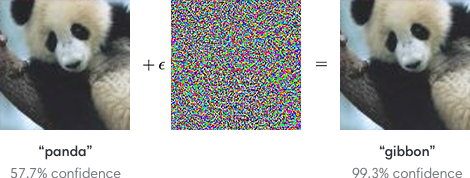As deep neural networks enter consumer and enterprise products of various forms, this brittleness can possibly have devastating consequences (Brown et al. 2018, Athalye et al. 2017, Evtimov & Eykholt et al. 2018, Li et al. 2019). Most strikingly, Tencent Keen Security Lab recently demonstrated that the neural network underlying Tesla Autopilot can be fooled by an adversarially crafted marker on the ground into swerving into the opposite lane.

Given the importance of the problem, many researchers have formulated security models of adversarial attacks, along with ways to defend against adversaries in such models. In the most popular security model in the academic circle today, the adversary is allowed to perturb an input by a small noise bounded in $\ell_p$-norm, in order to cause the network to misclassify it. Thus, given a loss function $L$, a norm bound $\epsilon$, an input $x$, its label $y$, and a neural network $F$, the adversary tries to find an input $\hat x$, within $\ell_p$-distance $\epsilon$ of $x$, that maximizes the loss $L(F(x), y)$, i.e. it solves the following optimization problem

$\hat x = \argmax_{\|x' - x\|_p \le \epsilon} L(F(x'), y).$

If $F$ has trainable parameters $\theta$, then the defense needs to find the parameters that minimizes $L(F(\hat x), y)$, for $(x, y)$ sampled from the data distribution $D$, i.e. it solves the following minimax problem

$\min_{\theta} \underset{(x, y) \sim D}{\mathbb{E}} L(F(\hat x), y).$

Empirically, during an attack, the adversarial input $\hat x$ can be obtained approximately by solving the max problem using gradient descent, making sure to project back to the $\epsilon$-ball after each step. This is known as the PGD attack (Kurakin et al., Madry et al.), short for “project gradient descent.” During training by the defense, for every sample $(x, y) \sim D$, this estimate of $\hat x$ can be plugged into the min problem for gradient descent of $\theta$. This is known as Adversarial Training, or PGD training specifically when PGD is used for finding $\hat x$.

## Empirical Robust Accuracy

Currently, the standard benchmark for measuring the strength of a model’s adversarial defense is the model’s (empirical) robust accuracy on various standard datasets like CIFAR-10 and Imagenet. This accuracy is calculated by attacking the model with a strong empirical attack (like PGD) for every sample of the test set. The percentage of the test set that the model is still able to correctly classify is the empirical robust accuracy.

For example, consider an adversary allowed to perturb an input by $\epsilon = \frac{8}{255}$ in $\ell_\infty$ norm. On an image, this means that the adversary can change the color of each pixel by at most 8 units (out of 255 total) in each color channel — a rather imperceptible perturbation. Currently, the state-of-the-art empirical robust accuracy against such an adversary on CIFAR-10 hovers around 55% (Zhang et al. 2019, Hendrycks et al. 2019), meaning that the best classifier can only withstand a strong attack on about 55% of the samples in CIFAR-10. Contrast this with the state-of-the-art nonrobust accuracy on CIFAR-10 of >95%. Thus it’s clear that adversarial robustness research still has a long way to go.

# Provable Robustness via Randomized Smoothing

Note that the empirical robust accuracy is only an upper bound on the true robust accuracy. This is defined by hypothetically replacing the strong empirical attack used in empirical robust accuracy with the ideal attack able to find $\hat x$ exactly for every $x$. Thus, nothing in principle prevents a stronger empirical attack from further lowering the empirical robust accuracy of a model. Indeed, except a few notable cases like PGD (Madry et al.), we have seen most claims of adversarial robustness broken down by systematic and thorough attacks (as examples, see Carlini & Wagner 2016, Carlini & Wagner 2017, Athalye et al. 2017, Uesato et al. 2018, Athalye et al. 2018, Engstrom et al. 2018, Carlini 2019).

This has motivated researchers into developing defenses that can certify the absence of adversarial examples (as prominent examples, see Wong & Kolter 2018, Katz et al. 2017, and see Salman et al. 2019 for a thorough overview of these techniques). Such a defense is afforded a provable (or certified) robust accuracy on each dataset, defined as the percentage of the test set that can be proved to have no adversarial examples in its neighborhood. In contrast with empirical robust accuracy, provable robust accuracy is a lower bound on the true robust accuracy, and therefore cannot be lowered further by more clever attacks. The tables in the beginning of our blog post, for example, display provable robust accuracies on CIFAR-10 and Imagenet.

Until recently, most such certifiable defenses have not been able to scale to large networks and datasets (Salman et al. 2019), but a new technique called randomized smoothing (Lecuyer et al., Li et al., Cohen et al.) was shown to bypass this limitation, obtaining highly-nontrival $\ell_2$ certified robust accuracy on Imagenet (Cohen et al.). We now briefly review randomized smoothing.

## Definition

Consider a classifier $f$ from $\mathbb{R}^d$ to classes $\mathcal{Y}$. Randomized smoothing is a method that constructs a new, smoothed classifier $g$ from the base classifier $f$. The smoothed classifier $g$ assigns to a query point $x$ the class which is most likely to be returned by the base classifier $f$ under isotropic Gaussian noise perturbation of $x$, i.e.,

$g(x) = \argmax_{c \in \mathcal{Y}} \; \mathbb{P}(f(x+\delta) = c)$

where $\delta \sim \mathcal{N}(0, \sigma^2 I)$, and the variance $\sigma^2$ is a hyperparameter of the smoothed classifier $g$ (it can be thought to control a robustness/accuracy tradeoff). In Cohen et al., $f$ is a neural network.

## Prediction

To estimate $g(x)$, one simply has to

1. Sample a collection of Gausian samples $\delta_i$.
2. Predict the class $y_i$ of each $x + \delta_i$ using the base classifier $f$.
3. Take the majority vote of the $y_i$’s as the final prediction of the smoothed classifier $g$ at $x$.

## Certification

The robustness guarantee presented by Cohen et al. is as follows: suppose that when the base classifier $f$ classifies $\mathcal{N}(x, \sigma^2 I)$, the (most popular) class $c_A$ is returned with probability $p_A = \mathbb{P}_\delta(f(x+\delta) = c_A)$, and the runner-up class $c_B$ is returned with probability $p_B = \max_{c \neq c_A} \mathbb{P}_\delta(f(x+\delta) = c)$. We estimate $p_A$ and $p_B$ using Monte Carlo sampling and confidence intervals1. Then the smoothed classifier $g$ is robust around $x$ within the radius

$\frac{\sigma}{2} \left(\Phi^{-1}(p_A) - \Phi^{-1}(p_B)\right),$

where $\Phi^{-1}$ is the inverse of the standard Gaussian CDF. Thus, the bigger $p_A$ is and the smaller $p_B$ is, the more provably robust $g$ is.

## Training

Cohen et al. simply trained the base classifier $f$ under Gaussian noise data augmentation with cross entropy loss, i.e. for each data point $(x, y)$, sample $\delta \sim \mathcal{N}(0, \sigma^2 I)$ and train $f$ on the example $(x+\delta, y)$. With this simple training regime applied to a Resnet-110 base classifier, they were able to obtain significant certified robustness on CIFAR-10 and Imagenet, as shown in our tables.

## An Illustration

The following figures modified from Cohen et al. illustrate randomized smoothing. The base classifier $f$ partitions the input space into different regions with different classifications, colored differently in the left figure. The regions’ Gaussian measures (under the Gaussian $\mathcal{N}(x, \sigma^2 I)$ whose level curves are shown as dashed lines) are shown as a histogram on the right. The class $c_A$ corresponding to the blue region is the output of the smoothed classifier $g(x)$; the class $c_B$ corresponding to the cyan region is the runner-up class. If $p_A$ is large enough and $p_B$ is small enough, then we can prove that $g(x') = c_A$ for all $\|x' - x\|_2 \le \epsilon$, i.e. $g$ is robust at $x$ for $\ell_2$ radius $\epsilon$.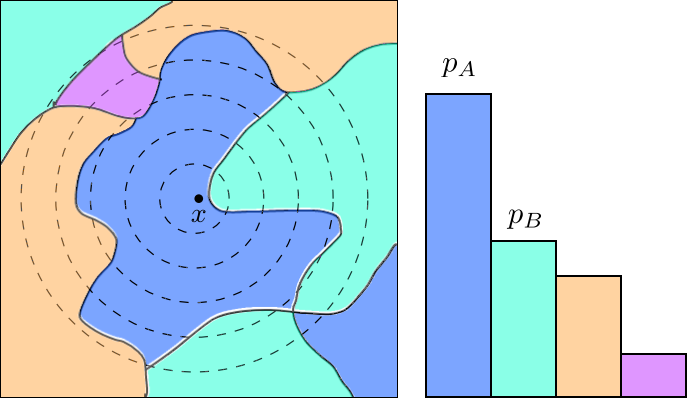# Adversarially Training the Smoothed Classifier

Intuitively, adversarial training attempts to make a classifier locally flat around input sampled from a data distribution. Thus it would seem that adversarial training should make it easier to certify the lack of adversarial examples, despite having no provable guarantees itself. Yet historically, it has been difficult to execute this idea (Salman et al. 2019, and folklore), with the closest being Xiao et al.

It is hence by no means a foregone conclusion that adversarial training should improve certified accuracy of randomized smoothing. A priori there could also be many ways these two techniques can be combined, and it is not clear which one would work best:

1. Train the base classifier $f$ to be adversarially robust, simultaneous with the Gaussian data augmentation training prescribed in Cohen et al..
2. Find an adversarial example of the base classifier $f$, then add Gaussian noise and train.
3. Add Gaussian noise and find an adversarial example of $f$ in the neighborhood of this Gaussian perturbation. Train $f$ on this adversarial example.
4. Find an adversarial example of the smoothed classifier $g$, then train $g$ on this example.

It turns out that certified accuracies of these methods follow the order (1) < (2) < (3) < (4), with (4) achieving the highest certified accuracies (see our paper). Indeed, in hindsight, if $g$ is the classifer doing the prediction, then we should be adversarially training $g$, and not $f$. In the rest of the blog post, we lay out the details of (4).

## Randomized Smoothing for Soft Classifiers

Neural networks typically learn soft classifiers, namely, functions $F: \mathbb{R}^d \to P(\mathcal{Y})$, where $P(\mathcal{Y})$ is the set of probability distributions over $\mathcal{Y}$. During prediction, the soft classifier is argmaxed to return the final hard classification. We therefore consider a generalization of randomized smoothing to soft classifiers. Given a soft classifier $F$, its associated smoothed soft classifier $G: \mathbb{R}^n \to P(\mathcal{Y})$ is defined as

$G (x) = \underset{\delta \sim \mathcal{N}(0, \sigma^2 I)}{\mathbb{E}} F(x + \delta).$

Let $f(x)$ and $F (x)$ denote the hard and soft classifiers learned by the neural network, respectively, and let $g$ and $G$ denote the associated smoothed hard and smoothed soft classifiers. Directly finding adversarial examples for the smoothed hard classifier $g$ is a somewhat ill-behaved problem because of the argmax, so we instead propose to find adversarial examples for the smoothed soft classifier $G$. Empirically we found that doing so will also find good adversarial examples for the smoothed hard classifier.

## Finding Adverarial Examples for Smoothed Soft Classifier

Given a labeled data point $(x, y)$, we wish to find a point $\hat x$ which maximizes the loss of $G$ in an $\ell_2$ ball around $x$ for some choice of loss function. As is canonical in the literature, we focus on the cross entropy loss $L_{\mathrm{CE}}$. Thus, given a labeled data point $(x, y)$ our (ideal) adversarial perturbation is given by the formula:

\begin{aligned} \hat x &= \argmax_{\|x' - x\|_2 \leq \epsilon} L_{\mathrm{CE} } (G (x'), y)\\ &= \argmax_{\|x' - x\|_2 \leq \epsilon} \left( - \log \underset{\delta \sim \mathcal{N} (0, \sigma^2 I)}{\mathbb{E}} F (x' + \delta)_y \right). \end{aligned}

We will refer to the above as the SmoothAdv objective. The SmoothAdv objective is highly non-convex, so as is common in the literature, we will optimize it via projected gradient descent (PGD), and variants thereof. It is hard to find exact gradients for SmoothAdv, so in practice we must use some estimator based on random Gaussian samples.

If we let $J(x') = L_{\mathrm{CE} } (G (x'), y)$ denote the SmoothAdv objective, then

$\nabla_{x'} J(x') = \nabla_{x'} \left( - \log \underset{\delta \sim \mathcal{N}(0, \sigma^2 I)}{\mathbb{E}} F (x' + \delta)_y \right) \; .$

However, it is not clear how to evaluate the expectation inside the log exactly, as it takes the form of a complicated high dimensional integral. Therefore, we will use Monte Carlo approximations. We sample i.i.d. Gaussians $\delta_1, \ldots, \delta_m \sim \mathcal{N} (0, \sigma^2 I)$, and use the plug-in estimator for the expectation:

$\nabla_{x'} J(x') \approx \nabla_{x'} \left( - \log \left( \frac{1}{m} \sum_{i = 1}^m F (x' + \delta_i)_y \right) \right) \; .$

It is not hard to see that if $F$ is smooth, this estimator will converge to $\nabla_{x'} J(x')$ as we take more samples.

## SmoothAdv is not the Naive Objective

We note that SmoothAdv should not be confused with the similar-looking objective

\begin{aligned} &\phantom{ {}={}} \argmax_{\|x' - x\|_2 \leq \epsilon} \underset{\delta \sim \mathcal{N} (0, \sigma^2 I)}{\mathbb{E}} L_{\mathrm{CE} } (F (x' + \delta), y) \\ &= \argmax_{\|x' - x\|_2 \leq \epsilon} \ \underset{\delta \sim \mathcal{N} (0, \sigma^2 I)}{\mathbb{E}} \left[-\log F(x' + \delta)_y\right] \; , \end{aligned}

where the $\log$ and $\mathbb{E}$ have been swapped compared to SmoothAdv, as suggested in section G.3 of Cohen et al. This objective, which we shall call naive, is the one that corresponds to finding an adversarial example of $F$ that is robust to Gaussian noise. In contrast, SmoothAdv directly corresponds to finding an adversarial example of $G$. From this point of view, SmoothAdv is the right optimization problem that should be used to find adversarial examples of $G$. This distinction turns out to be crucial in practice: empirically, Cohen et al found attacks based on the naive objective not to be effective. In our paper, we perform SmoothAdv-attack on Cohen et al.’s smoothed model and find, indeed, that it works better than the Naive objective, and it performs better with more Gaussian noise samples used to estimate its gradient.

We now wish to use our new SmoothAdv attack to boost the adversarial robustness of smoothed classifiers. As described in the beginning of this blog post, in (ordinary) adversarial training, given a current set of model parameters $\theta_t$ and a labeled data point $(x_t, y_t)$, one finds an adversarial perturbation $\hat x_t$ of $x_t$ for the current model, and then takes a gradient step for the model parameters $\theta_t$, evaluated at the point $(\hat x_t, y_t)$. Intuitively, this encourages the network to learn to minimize the worst-case loss over a neighborhood around the input.

What is different in our proposed algorithm is that we are finding the adversarial example $\hat x_t$ with respect to the smoothed classifier $G$ using the SmoothAdv objective, and we are training $G$ at this adversarial example $\hat x_t$ with respect to the SmoothAdv objective, estimated by the plug-in estimator.

\begin{aligned} \theta_{t+1} &= \theta_t + \eta \nabla_\theta \log\left(\frac{1}{m'} \sum_{i=1}^{m'} F(\hat x_t + \delta_i)_y\right), \end{aligned}

where $\theta_t$ are the parameters of $F$ at time $t$, $\delta_i \sim \mathcal{N}(0, \sigma^2 I)$, and $\eta$ is a learning rate.

## More Data: Pretraining and Semisupervision

Hendrycks et al. showed that pre-training on Imagenet can improve empirical adversarial robustness on CIFAR-10 and CIFAR-100. Similarly, Carmon et al. showed that augmenting supervised adversarial training with unsupervised training on a carefully selected unlabeled dataset confers significant robustness improvement. We adopt these ideas for randomized smoothing and confirm that these techniques are highly beneficial for certified robustness as well, especially for smaller radii (though unfortunately their combination did not induce combined improvement). See the tables below.

## Results

Over the course of the blog post, we have introduced several hyperparameters, such as 1) $\epsilon$, the radius of perturbation used for adversarial training, 2) $m$, the number of Gaussian noise samples, 3) $\sigma$, the standard deviation of the Gaussian noise. We also did not mention other hyperparameters like $T$, the number of iterations used for PGD iterations, or the usage of DDN, an alternative attack to PGD that has been shown to be effective for $\ell_2$-perturbations (Rony et al.). In our paper we do extensive analysis of the effects of these hyperparameters, to which we refer interested readers.

Taking the max over all such hyperparameter combinations for each $\ell_2$ perturbation radius, we obtain the upper envelopes of the certified accuracies of our method vs the upper envelopes of Cohen et al. in the tables in the beginning of this post, which we also replicate here for convenience.

$\ell_2$ radius (Imagenet) 0.5 1 1.5 2 2.5 3 3.5
Cohen et al. (%) 49 37 29 19 15 12 9
Ours (%) 56 43 37 27 25 20 16
$\ell_2$ radius (CIFAR-10) 0.25 0.5 0.75 1.0 1.25 1.5 1.75 2.0 2.25
Cohen et al. (%) 60 43 32 23 17 14 12 10 8
Ours (%) 74 57 48 38 33 29 24 19 17
+ Pre-training (%) 80 63 52 39 36 30 25 20 17
+ Semi-supervision (%) 80 63 53 39 36 32 25 20 18
+ Both (%) 82 65 52 38 34 30 25 21 18

# Conclusion

In this blog post, we reviewed adversarial training and randomized smoothing, a recently proposed provable defense. By adversarially training the smoothed classifier — and carefully getting all the details right — we obtained the state-of-the-art $\ell_2$ provable robustness on CIFAR-10 and Imagenet, demonstrating significant improvement over randomized smoothing alone.

# Acknowledgements

This blog post presented work done by Hadi Salman, Greg Yang, Jerry Li, Huan Zhang, Pengchuan Zhang, Ilya Razenshteyn, and Sebastien Bubeck. We would like to thank Zico Kolter, Jeremy Cohen, Elan Rosenfeld, Aleksander Madry, Andrew Ilyas, Dimitris Tsipras, Shibani Santurkar, Jacob Steinhardt for comments and discussions during the making of this paper.

1. We actually estimate a lower bound $\underline{p_A}$ of $p_A$ and an upper bound $\overline{p_B}$ of $p_B$ with high probability, and substitute $\underline{p_A}$ and $\overline{p_B}$ for $p_A$ and $p_B$ everywhere. This is an overestimate, so our guarantee holds except for a small probability that the estimates are wrong. See Cohen et al. or our paper for more details.

]]>